81% OFF online library of tutorials for mastering Google Sheets

One time purchase \$199

Hey there! Register a new account to access the full suite of features, tutorials, and more..

# VLOOKUP

Use VLOOKUP() when you want a specific value in a column of data. Use VLOOKUP() when you want to retrieve information from a different column based on that value. Great for creating amazing summaries, dynamic reports, or managing inventory

# SUMIF

When you need to calculate the sum of values in a range of cells that meet specific criteria, use SUMIF(). Great to use when you need to filter data. For instance, you can use it to sum all the revenue generated from a particular region, all the orders placed by a specific customer, or all the products with a certain attribute.

# COUNTIF

Use COUNTIF() when you're trying to figure out frequency. Learn how to use COUNTIF in Google Sheets. Also check out it's possible alternative: SUMIF. Depending on what you're trying to do, COUNTIF could be your solution or not.

# IF

Powerful and mysterious. Use IF() to make your spreadsheets gateways. Learn how to use IF in Google Sheets. Also check out it's oft-partner ISBLANK()

# FILTER

the FILTER() formula in Google Sheets allows you to filter data based on a set of criteria. Learn how to use FILTER in Google Sheets. Also check out it's possible combination alternative: INDEX/SORT.

# INDEX

A seemingly innocent and useless formula can be so powerful. INDEX pairs nicely with MATCH to replace VLOOKUP. And it's uses are almost endless. Learn more about the INDEX() formula in Google Sheets.

# VLOOKUP

Powerful vlookup and easy to use. Learn how to use VLOOKUP in Google Sheets. Also check out it's possible alternative: INDEX/MATCH.

# SUMIF

Don't know the syntax, or how to use SUMIF? Learn how to use SUMIF in Google Sheets. Also check out it's possible alternative: COUNTIF. Depending on what you're trying to do, SUMIF could be your solution or not.

# CONCATENATE

Probably the most easily misspelled formula in all of Google Sheets. Learn how to use CONCATENATE in Google Sheets. Also check out it's possible alternative: JOIN.

# 515Formulas

Every Formula in Google Sheets, From ABS to ZTEST, including Vlookup and, my favorite, IF()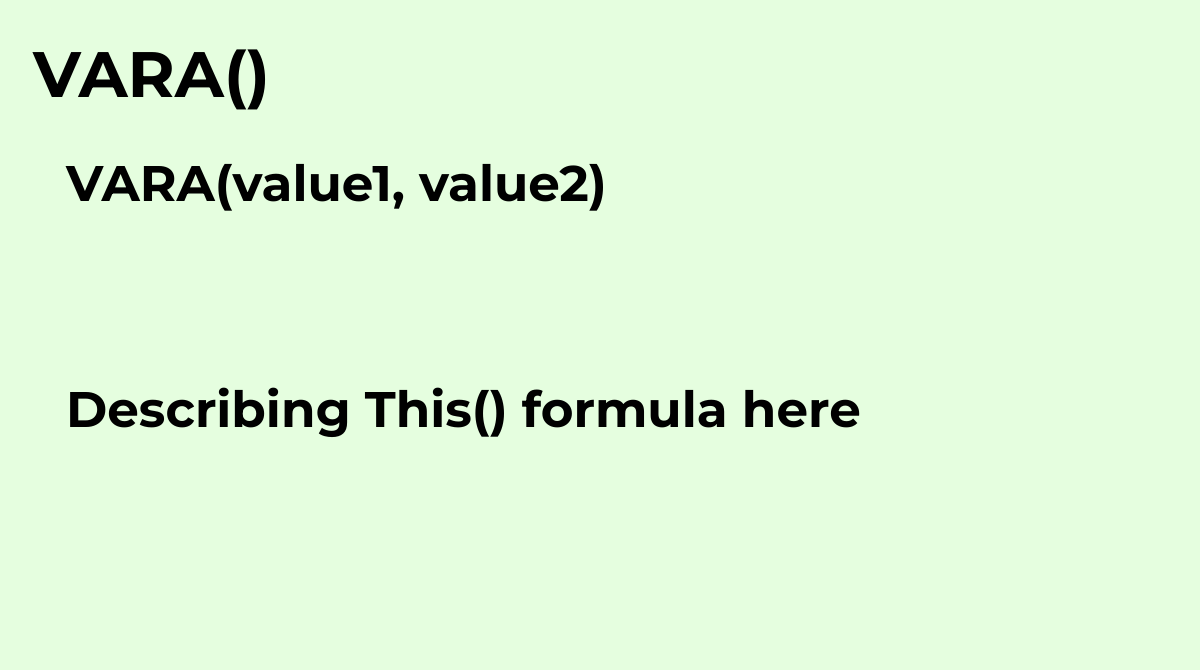Calculates an estimate of variance based on a sample, setting text to the value `0`.
`VARA(value1, value2)`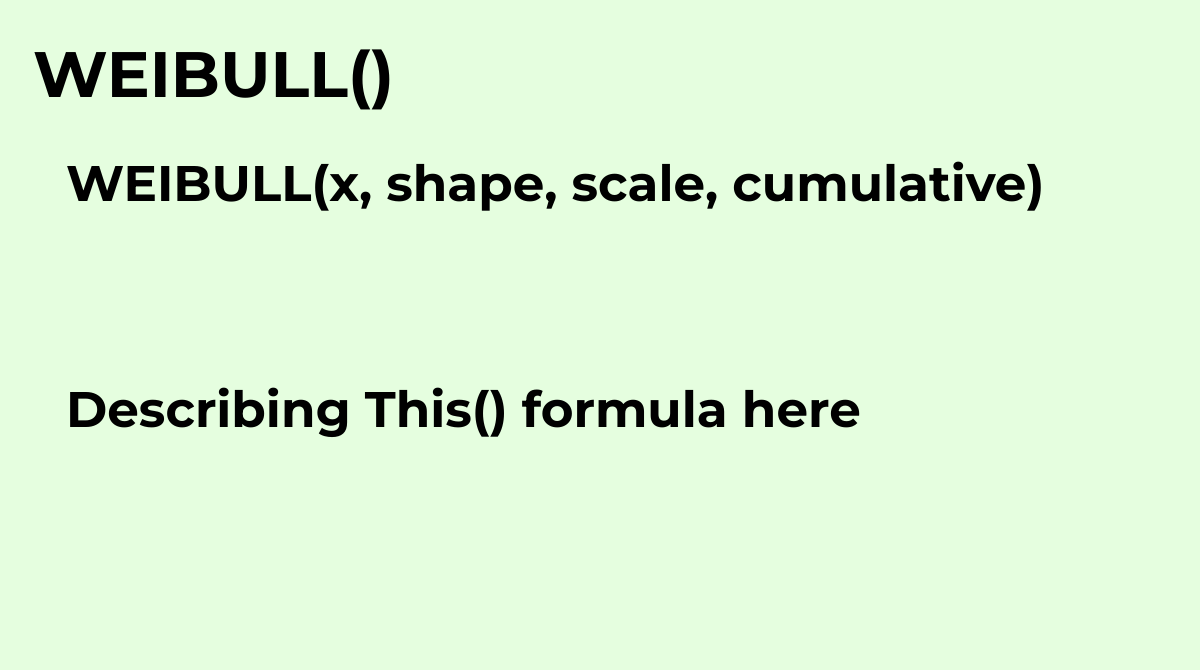Returns the value of the Weibull distribution function (or Weibull cumulative distribution functi...
`WEIBULL(x, shape, scale, cumulative)`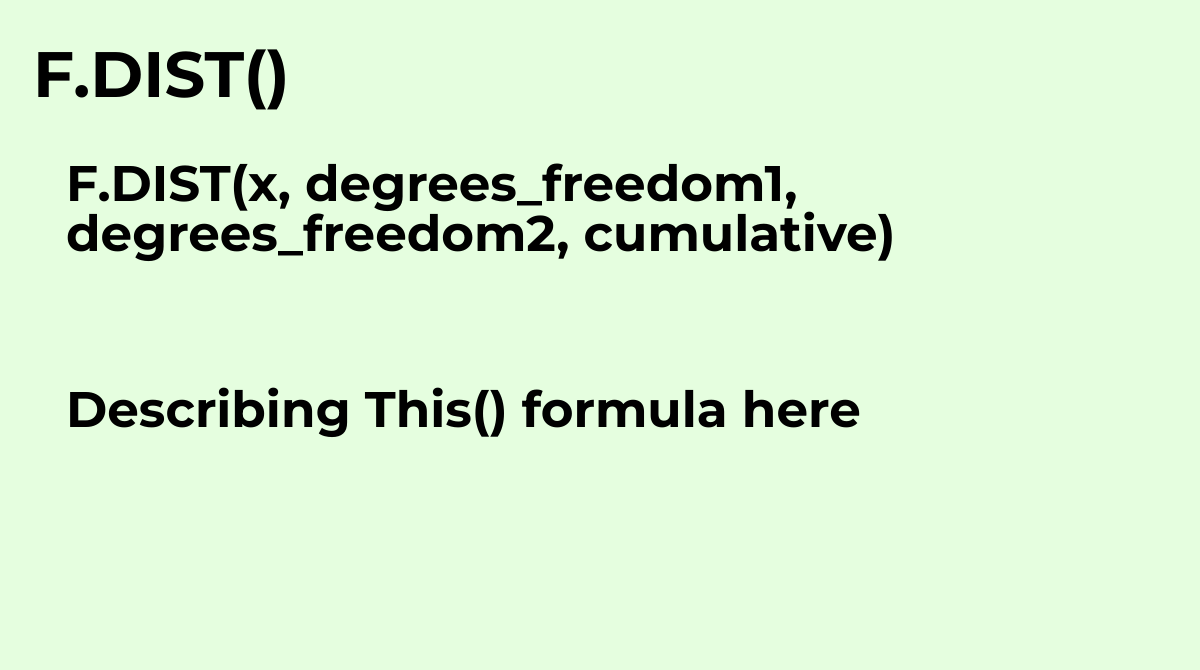Calculates the left-tailed F probability distribution (degree of diversity) for two data sets wit...
`F.DIST(x, degrees_freedom1, degrees_freedom2, cumulative)`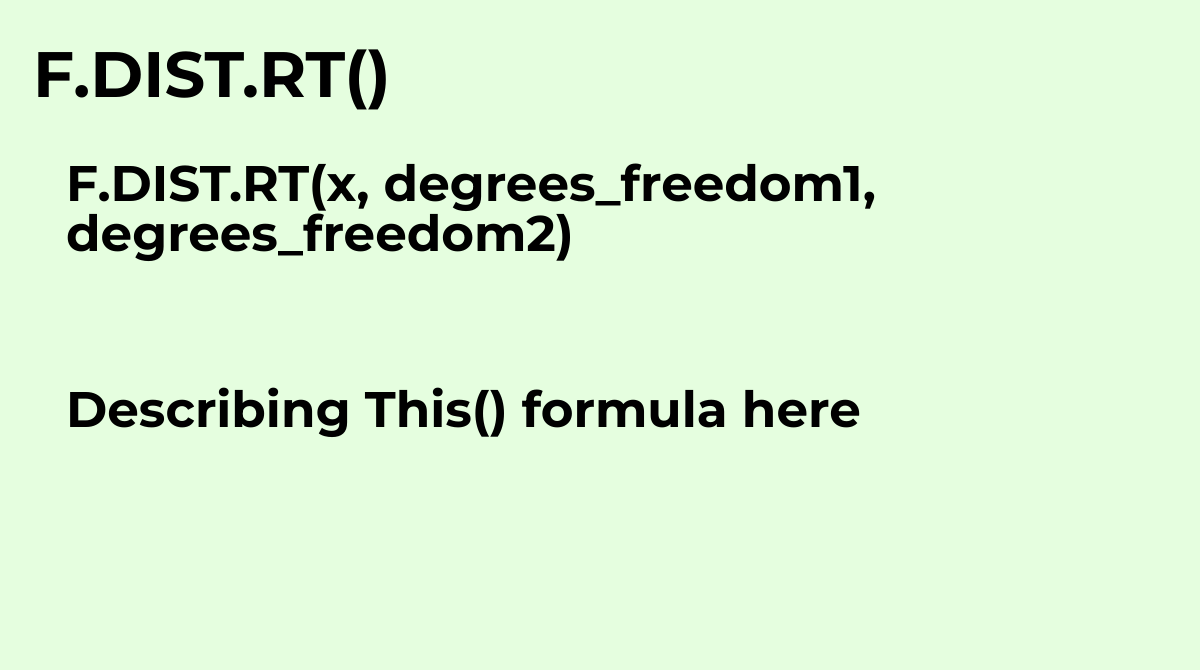Calculates the right-tailed F probability distribution (degree of diversity) for two data sets wi...
`F.DIST.RT(x, degrees_freedom1, degrees_freedom2)`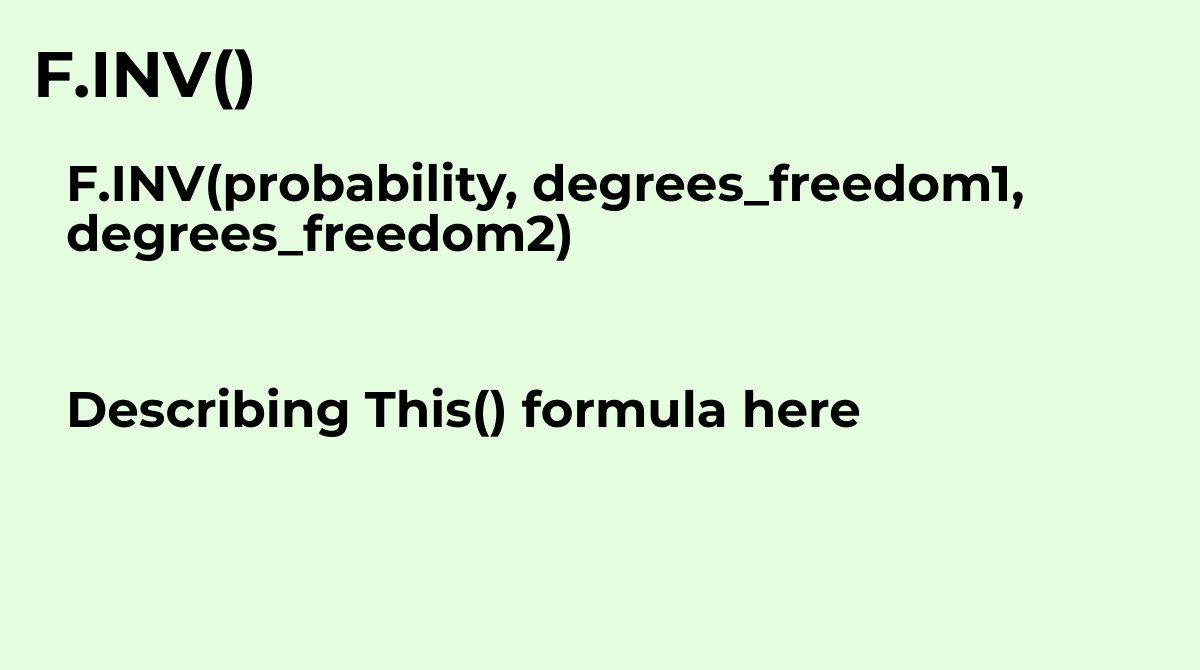Calculates the inverse of the left-tailed F probability distribution. Also called the Fisher-Sned...
`F.INV(probability, degrees_freedom1, degrees_freedom2)`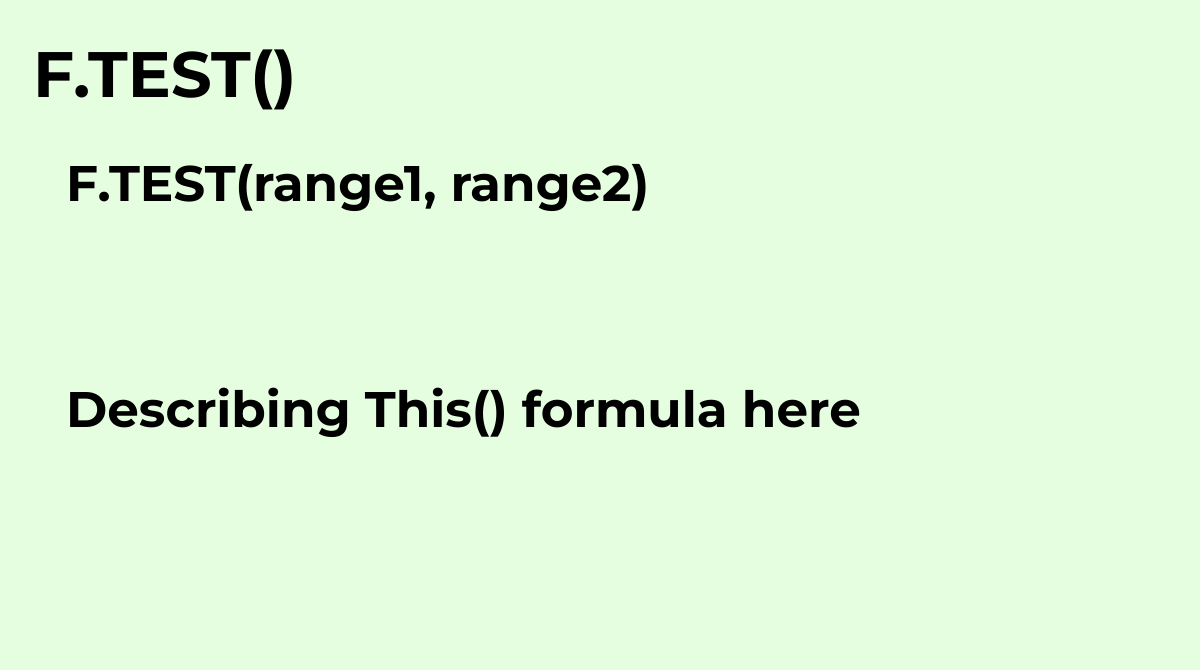See FTEST.
`F.TEST(range1, range2)`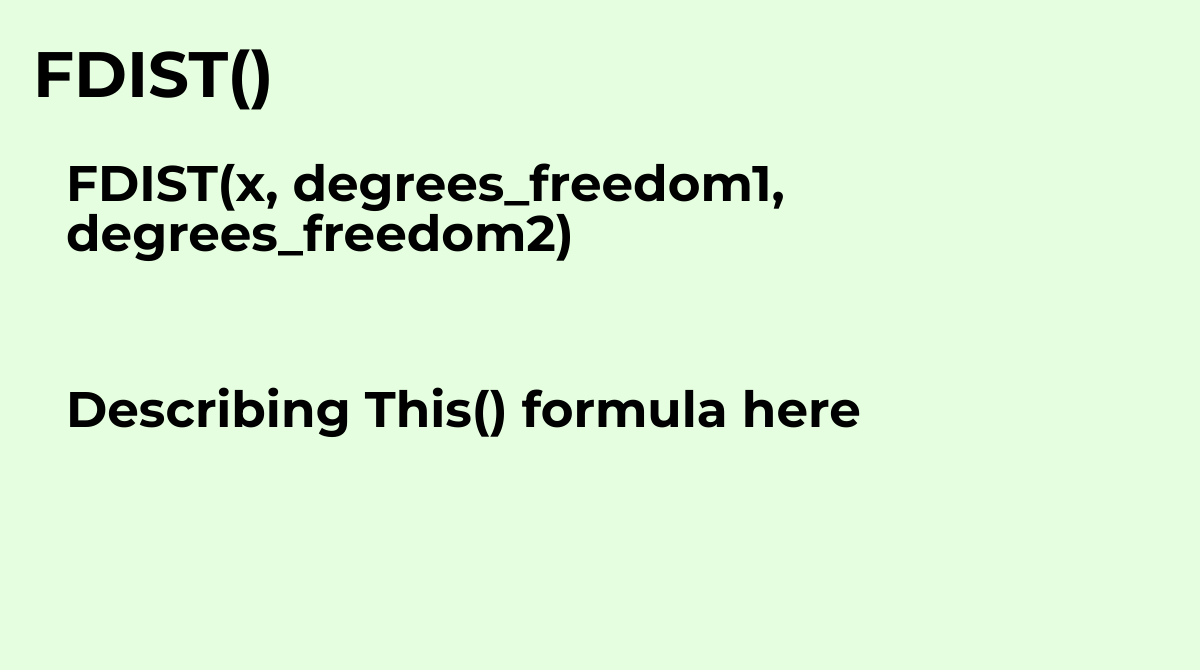See F.DIST.RT.
`FDIST(x, degrees_freedom1, degrees_freedom2)`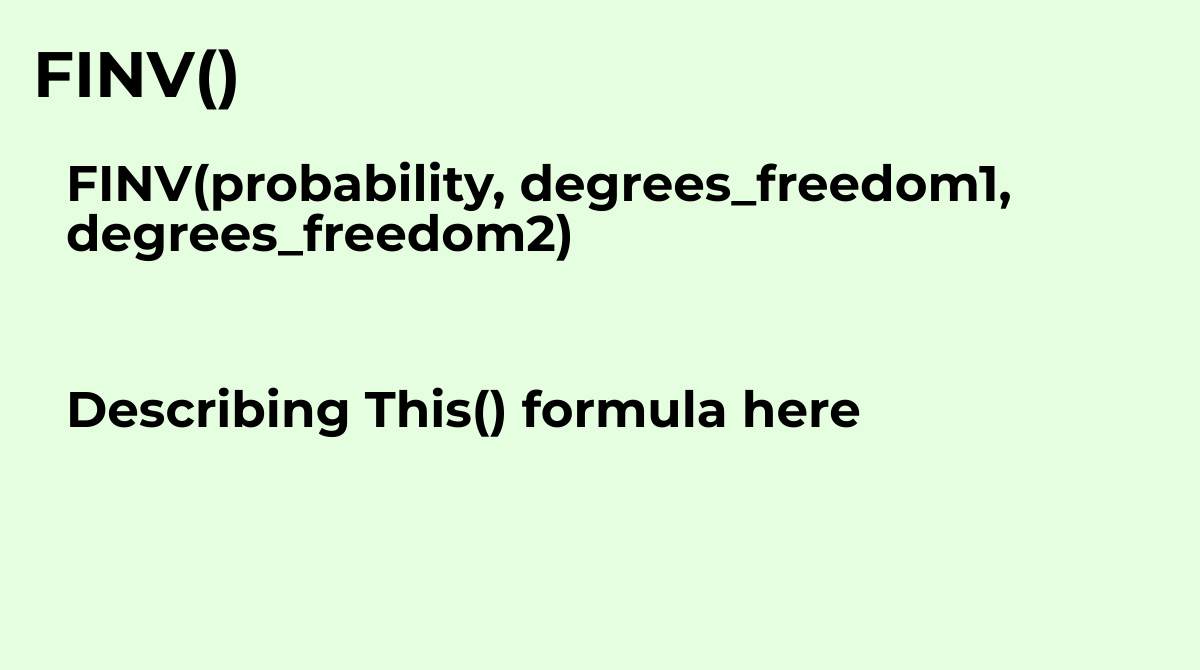See F.INV.RT
`FINV(probability, degrees_freedom1, degrees_freedom2)`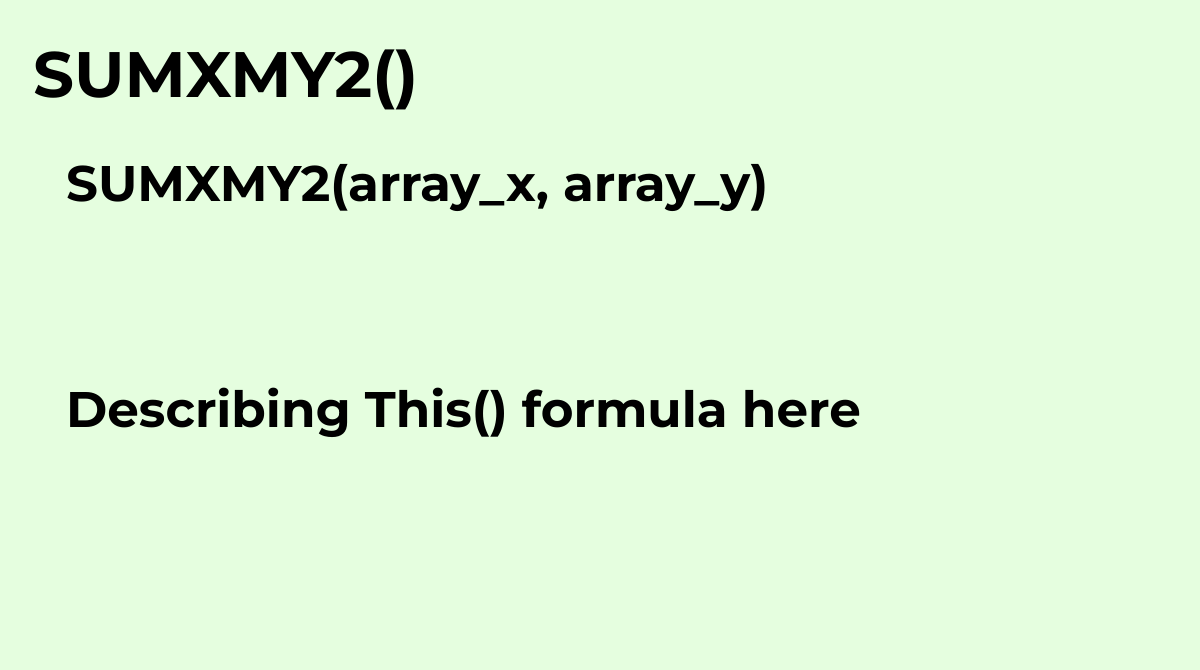Calculates the sum of the squares of differences of values in two arrays.
`SUMXMY2(array_x, array_y)`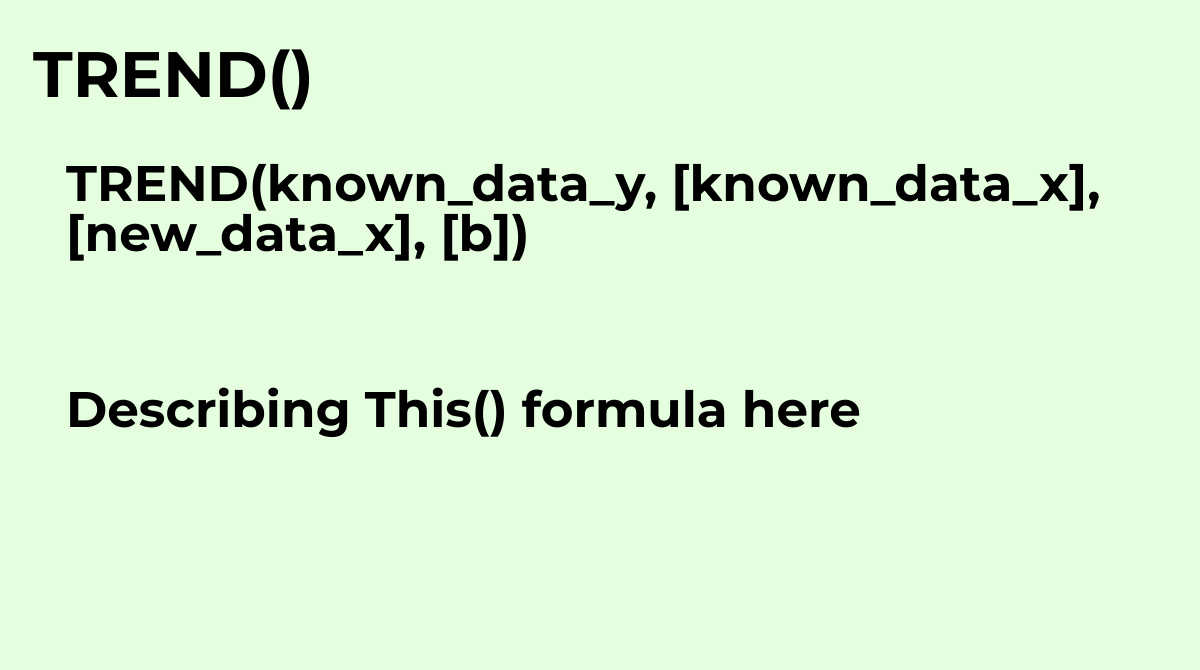Given partial data about a linear trend, fits an ideal linear trend using the least squares metho...
`TREND(known_data_y, [known_data_x], [new_data_x], [b])`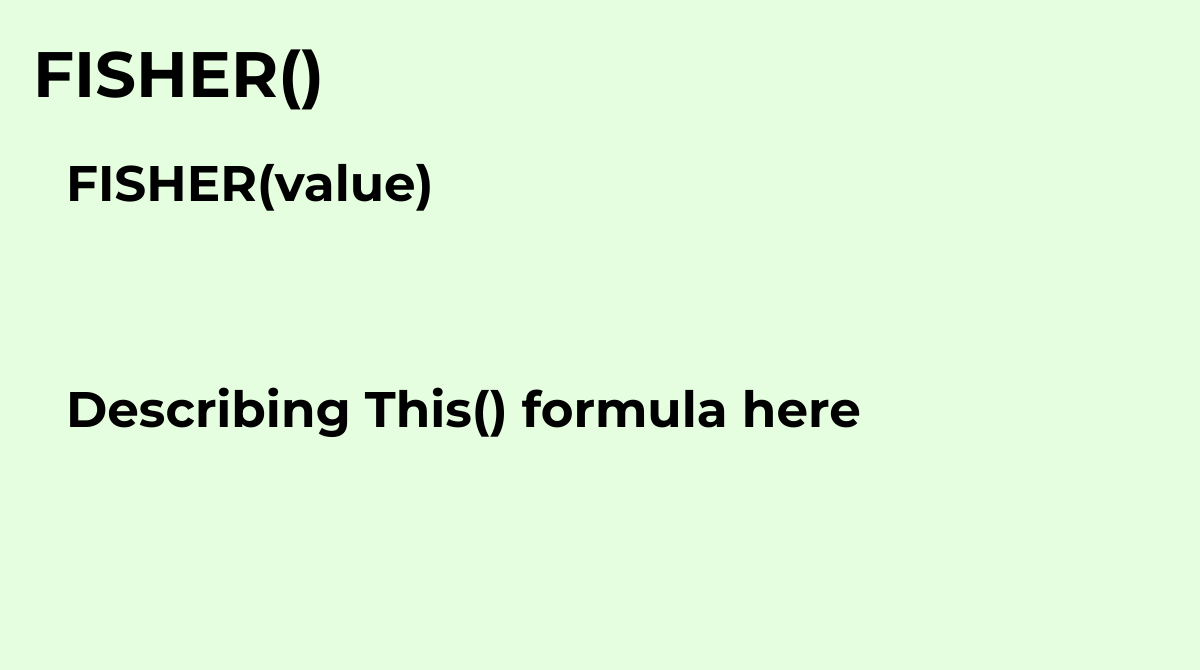Returns the Fisher transformation of a specified value.
`FISHER(value)`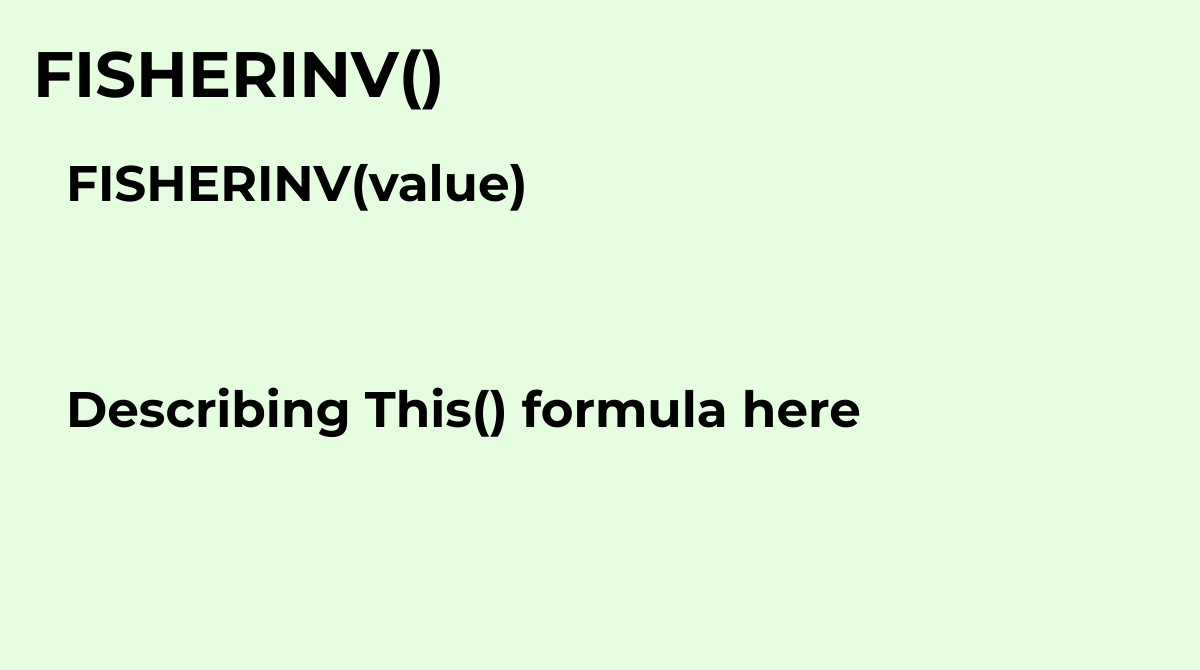Returns the inverse Fisher transformation of a specified value.
`FISHERINV(value)`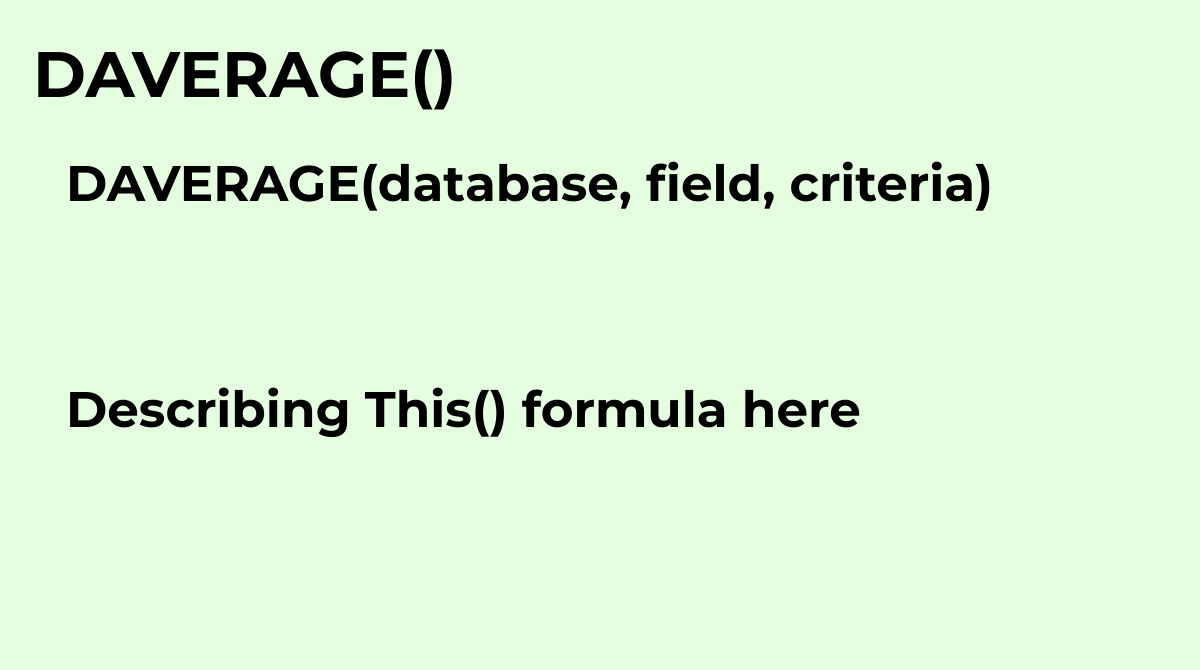Returns the average of a set of values selected from a database table-like array or range using a...
`DAVERAGE(database, field, criteria)`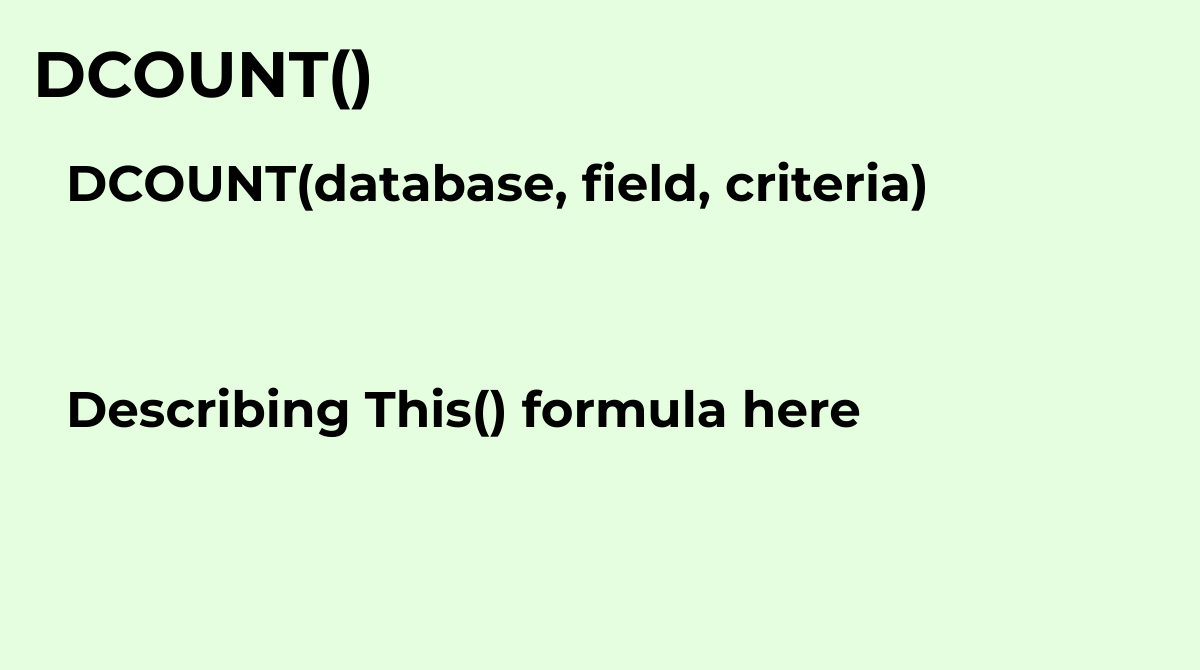Counts numeric values selected from a database table-like array or range using a SQL-like query.
`DCOUNT(database, field, criteria)`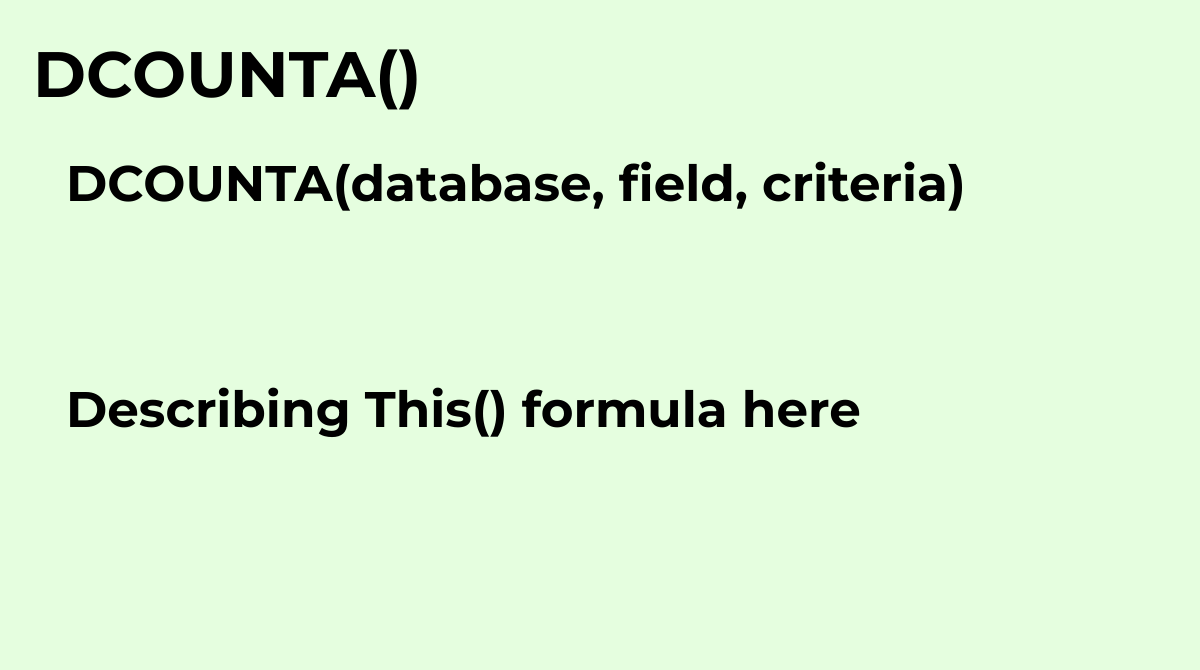Counts values, including text, selected from a database table-like array or range using a SQL-lik...
`DCOUNTA(database, field, criteria)`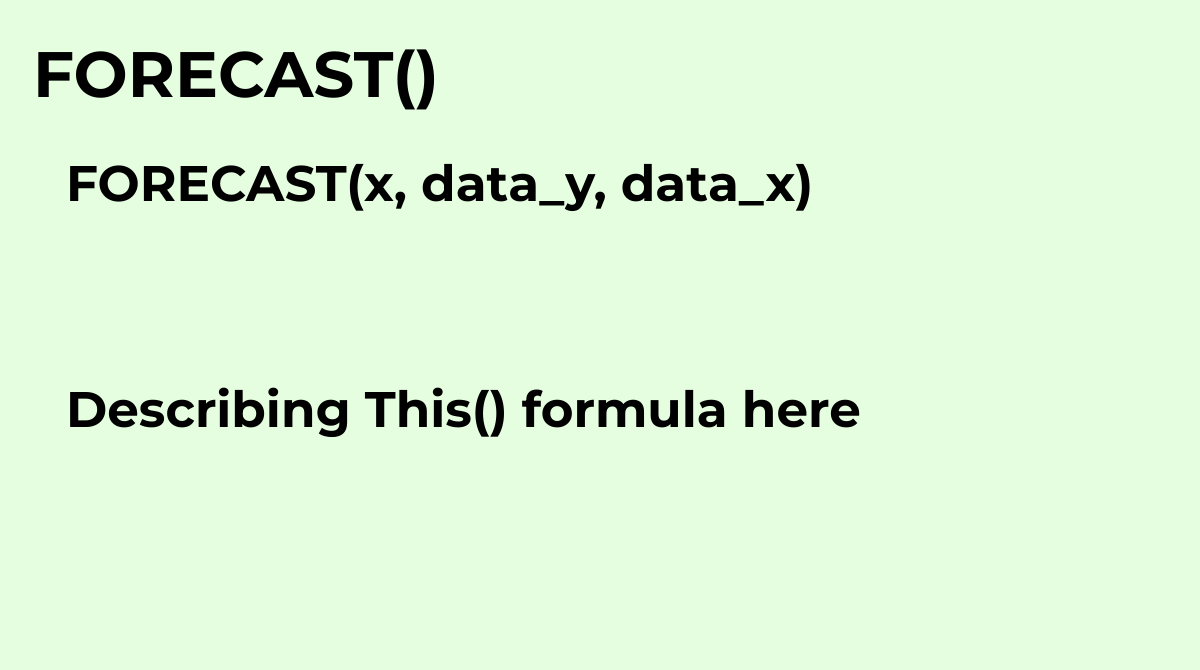Calculates the expected y-value for a specified x based on a linear regression of a dataset.
`FORECAST(x, data_y, data_x)`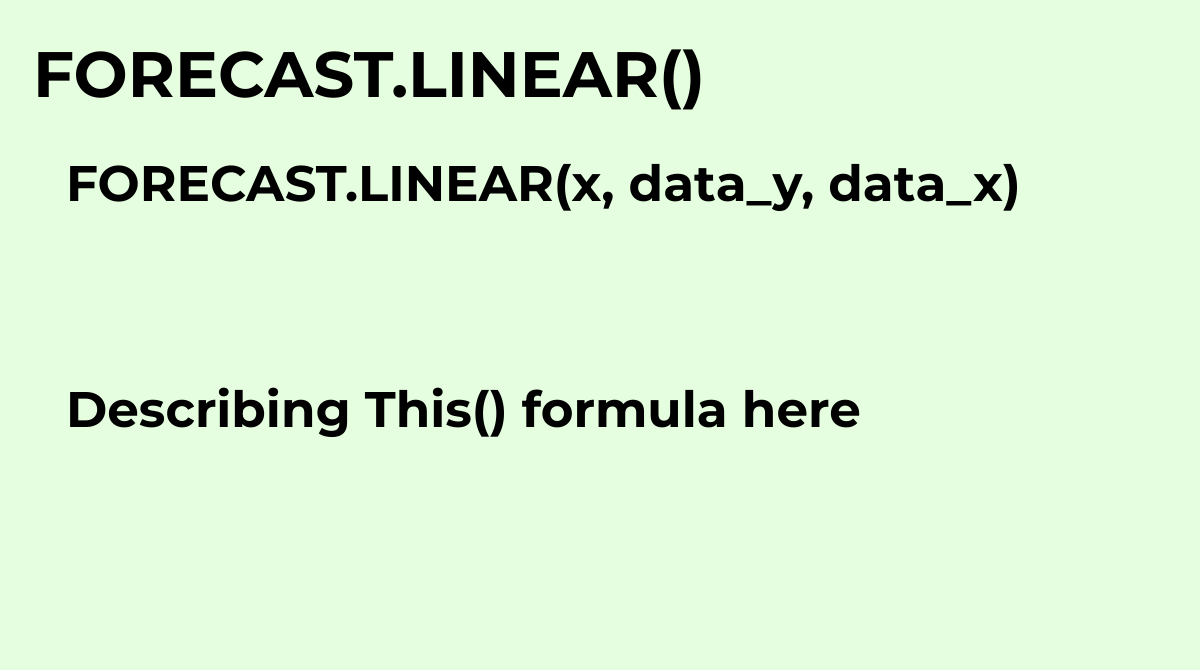See FORECAST
`FORECAST.LINEAR(x, data_y, data_x)`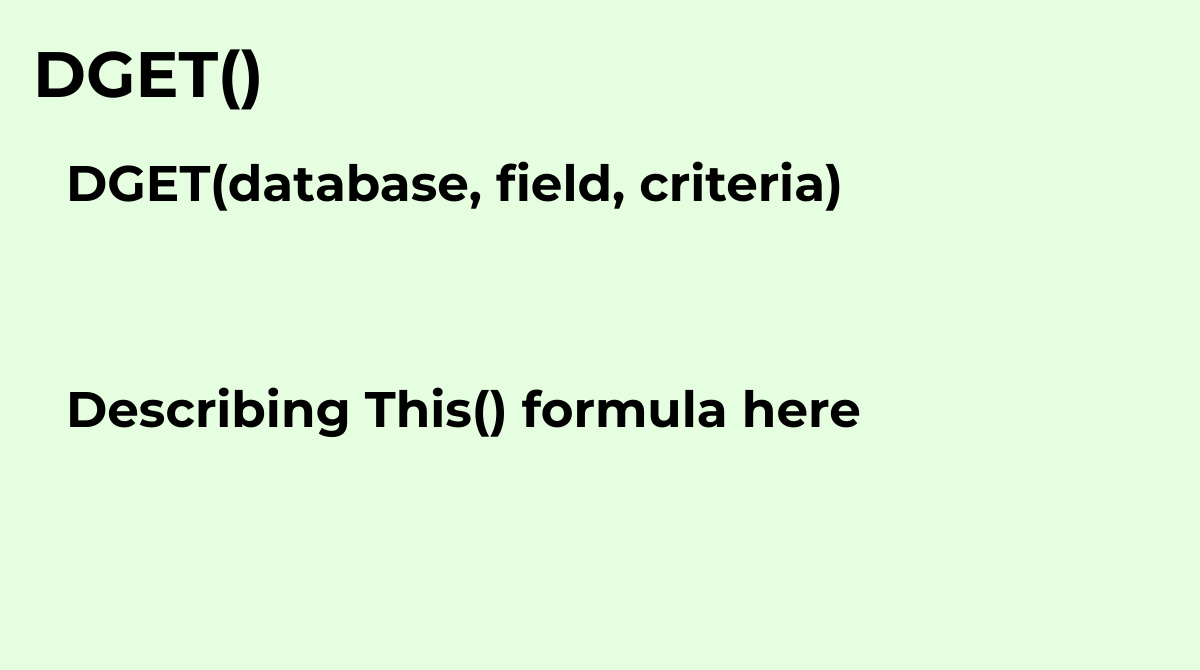Returns a single value from a database table-like array or range using a SQL-like query.
`DGET(database, field, criteria)`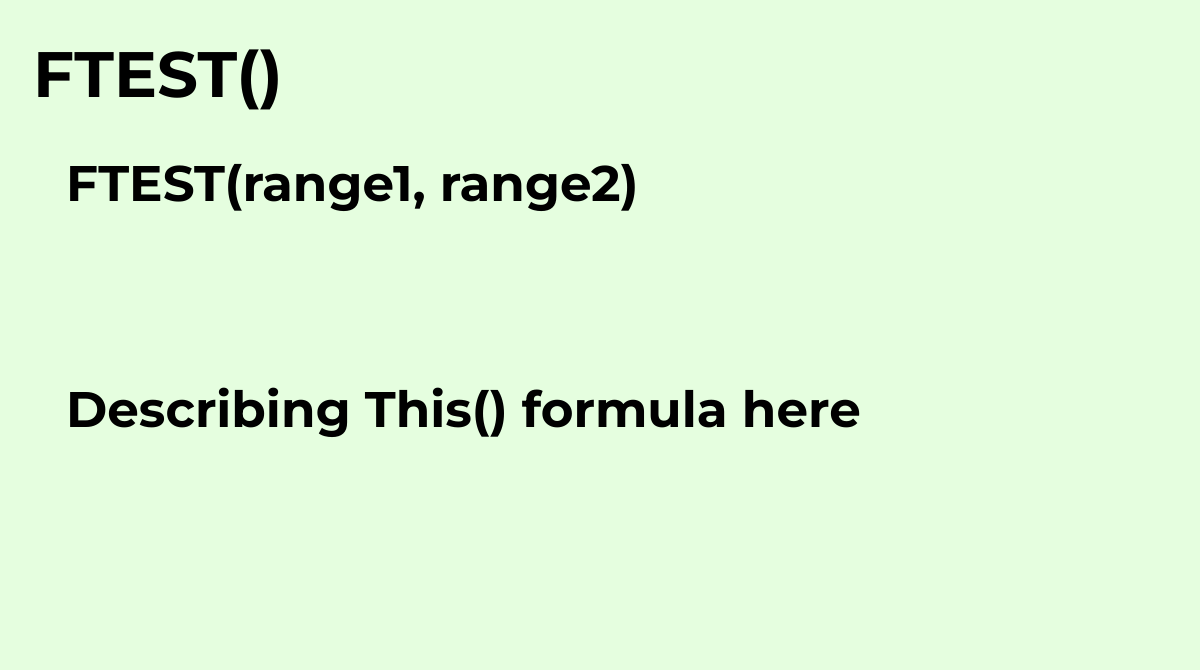Returns the probability associated with an F-test for equality of variances. Determines whether t...
`FTEST(range1, range2)`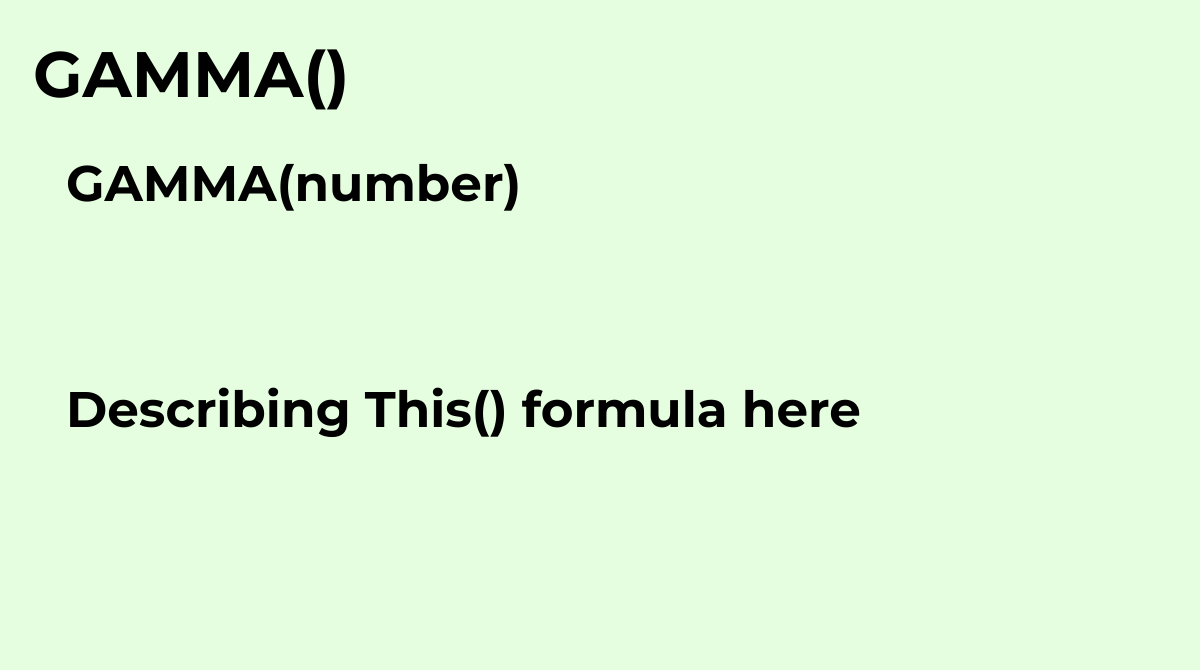Returns the Gamma function evaluated at the specified value..
`GAMMA(number)`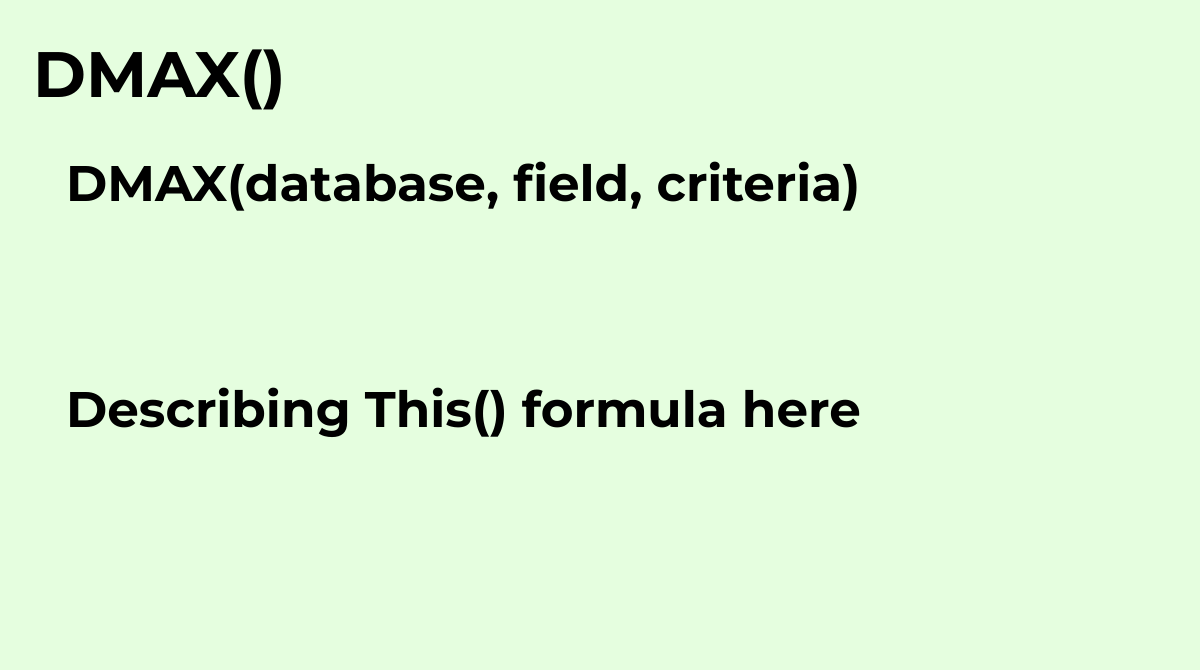Returns the maximum value selected from a database table-like array or range using a SQL-like query.
`DMAX(database, field, criteria)`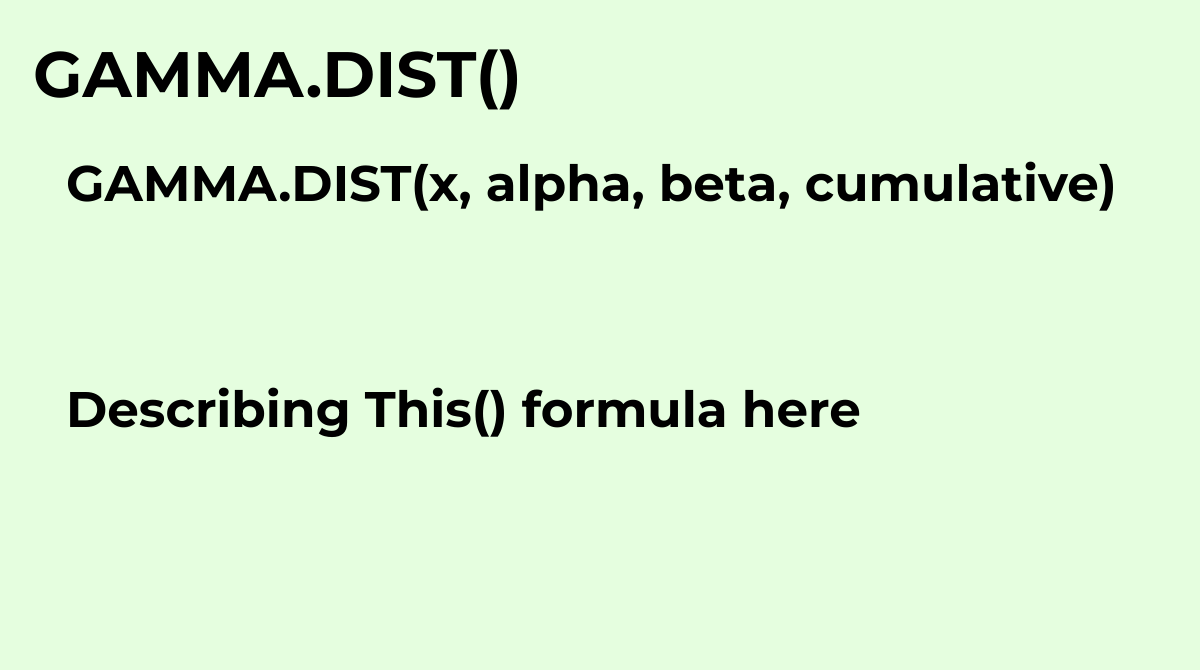Calculates the gamma distribution, a two-parameter continuous probability distribution.
`GAMMA.DIST(x, alpha, beta, cumulative)`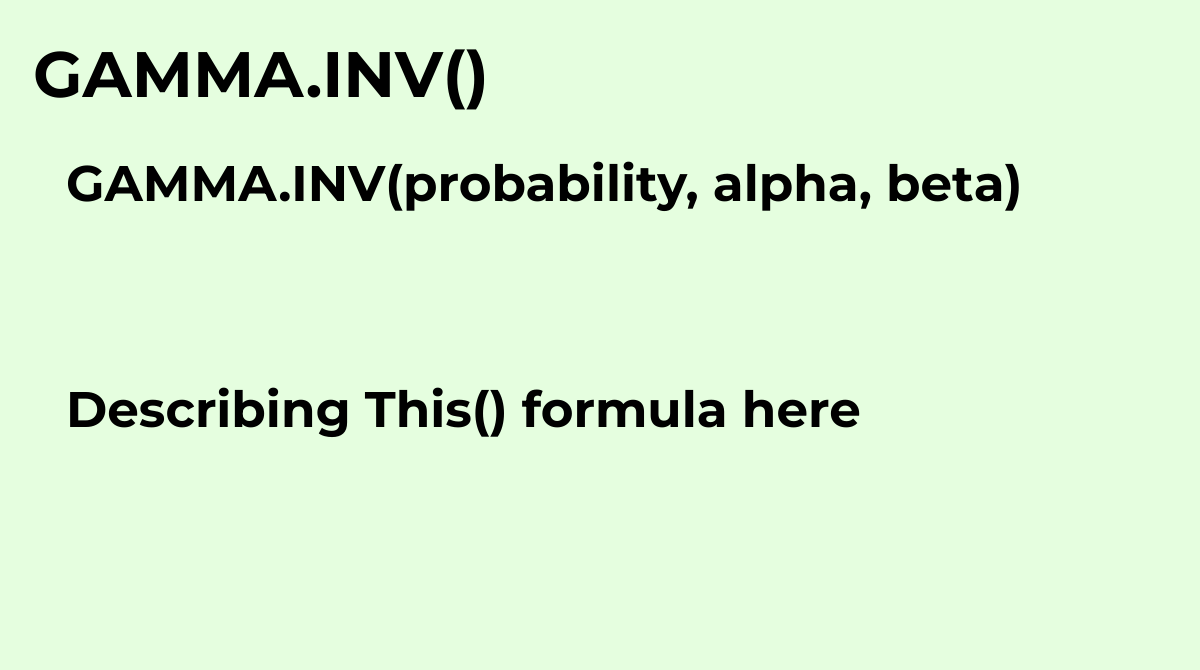The GAMMA.INV function returns the value of the inverse gamma cumulative distribution function fo...
`GAMMA.INV(probability, alpha, beta)`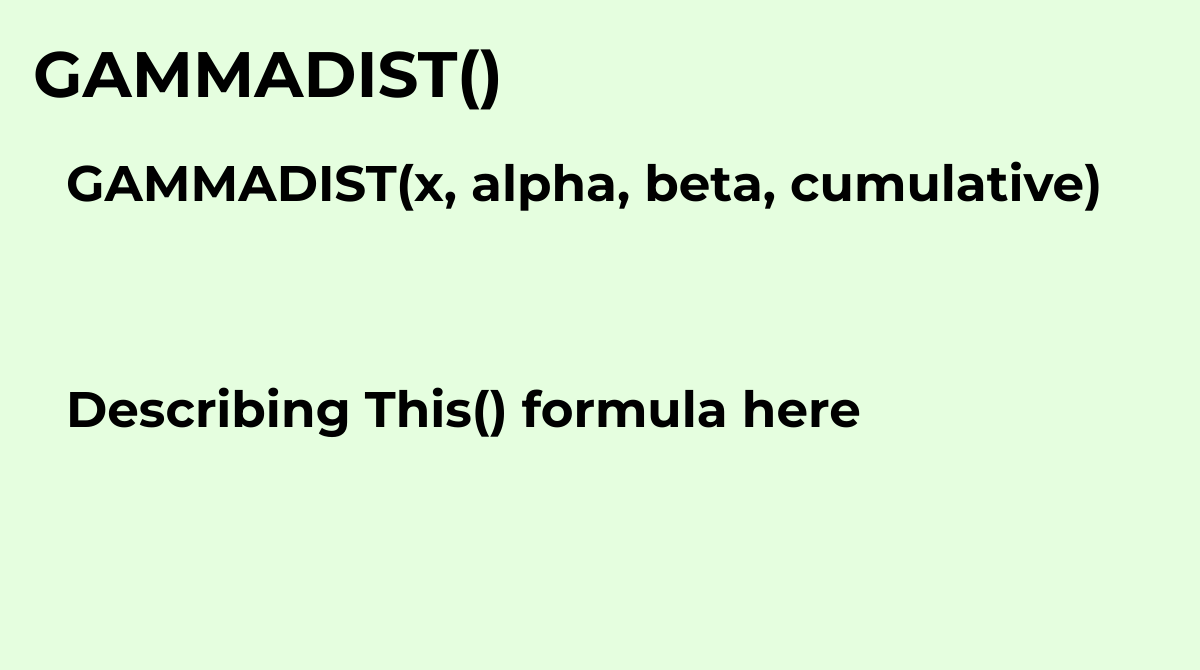See GAMMA.DIST
`GAMMADIST(x, alpha, beta, cumulative)`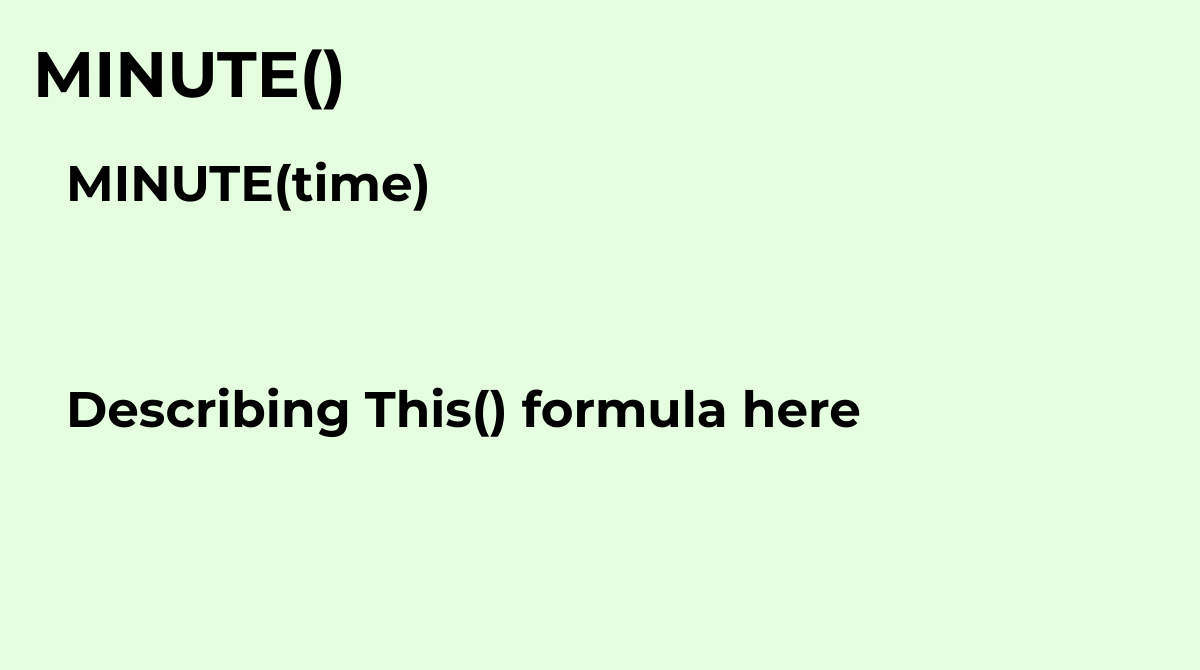Returns the minute component of a specific time, in numeric format.
`MINUTE(time)`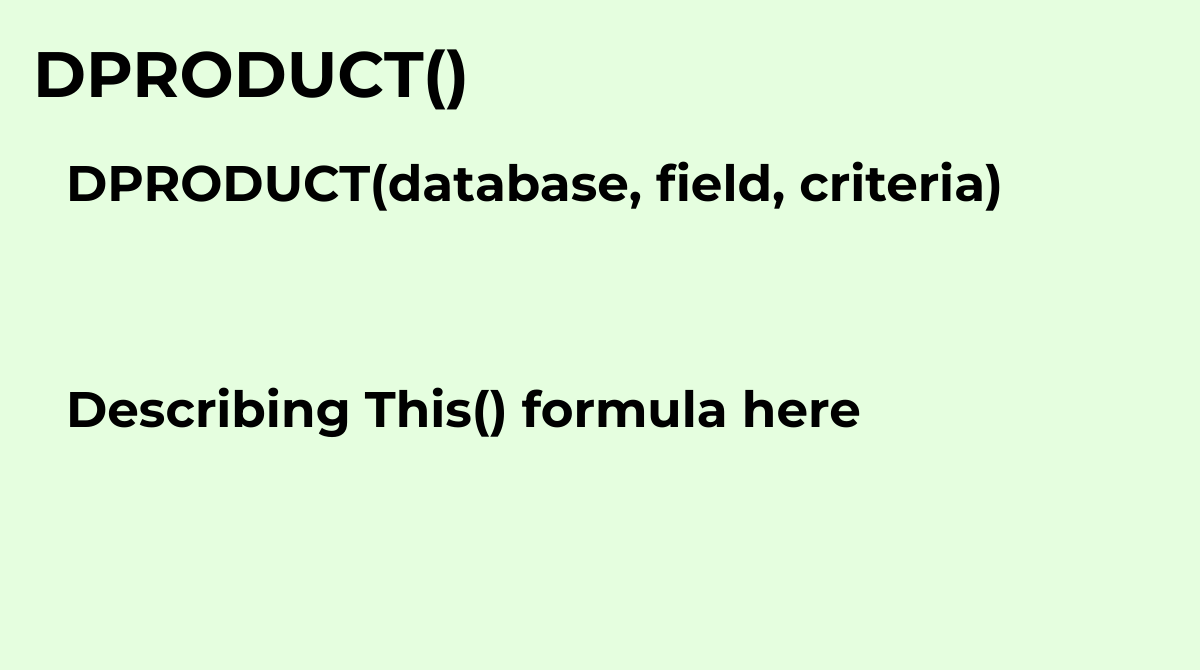Returns the product of values selected from a database table-like array or range using a SQL-like...
`DPRODUCT(database, field, criteria)`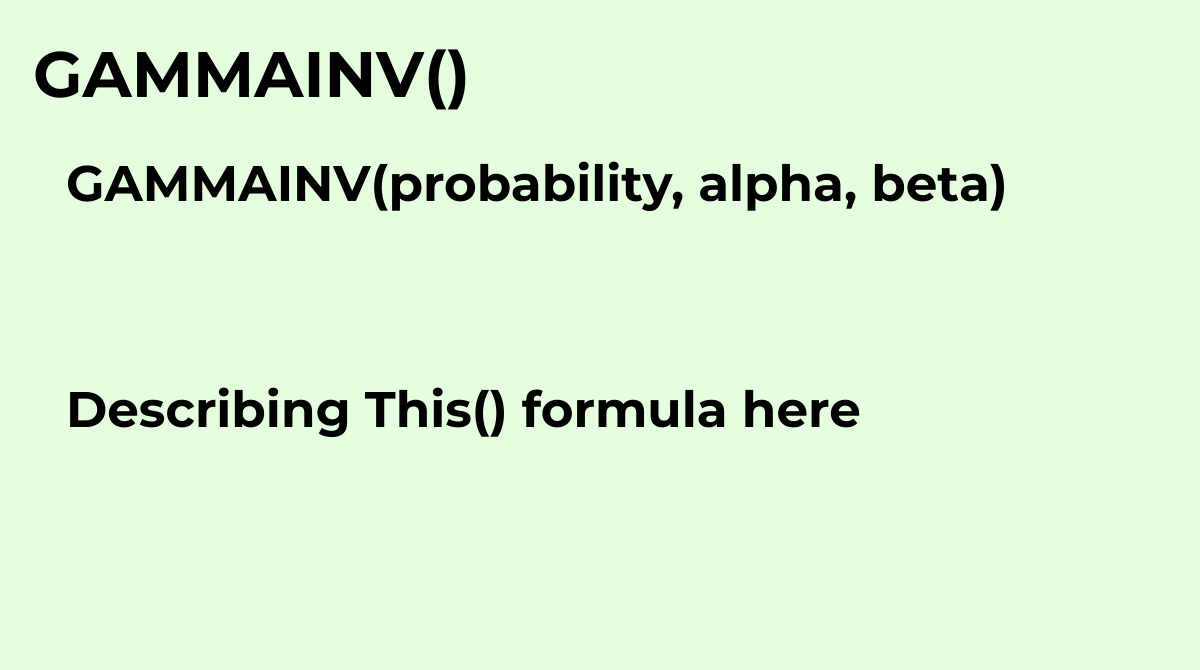See GAMMA.INV.
`GAMMAINV(probability, alpha, beta)`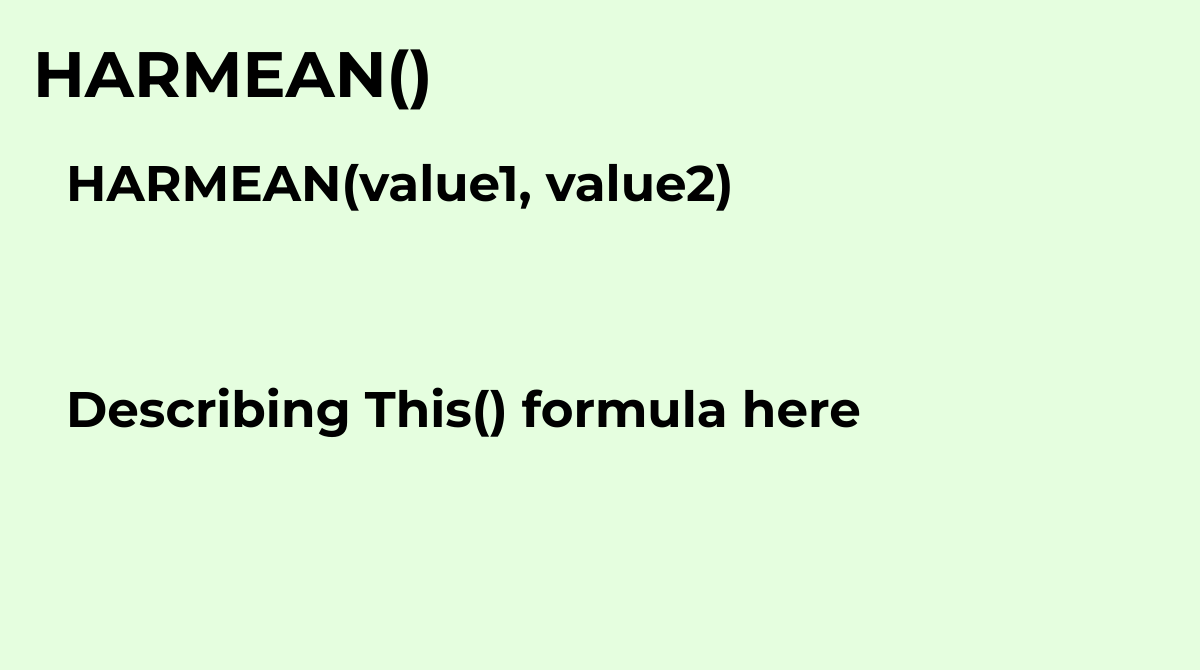Calculates the harmonic mean of a dataset.
`HARMEAN(value1, value2)`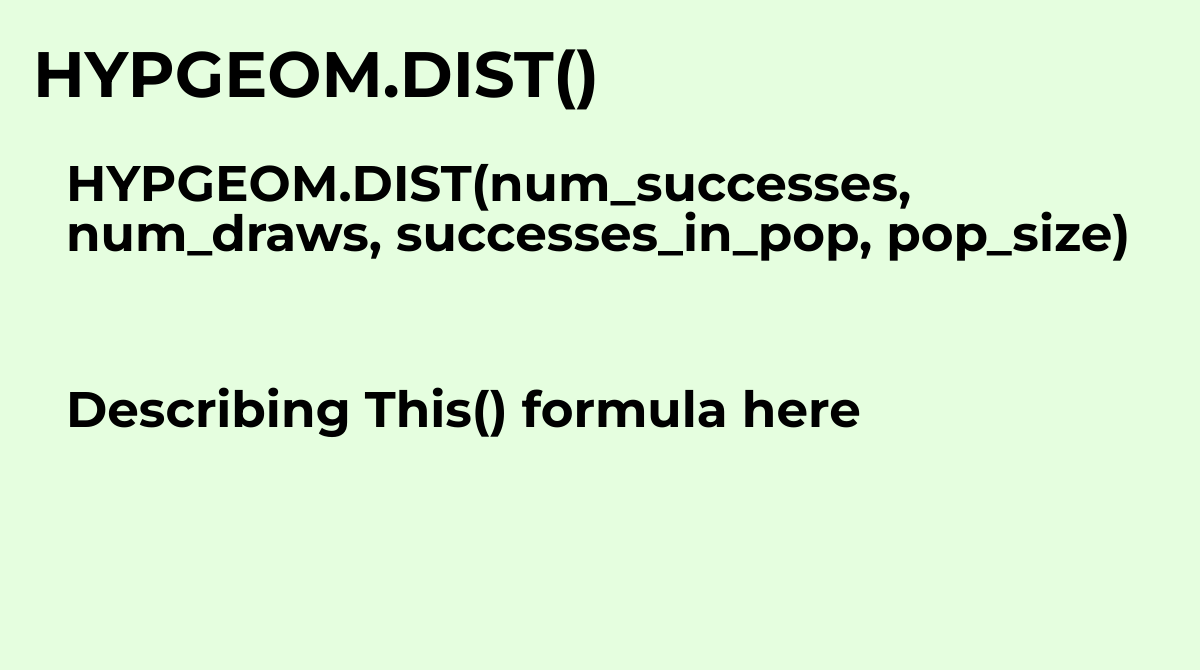See HYPGEOMDIST
`HYPGEOM.DIST(num_successes, num_draws, successes_in_pop, pop_size)`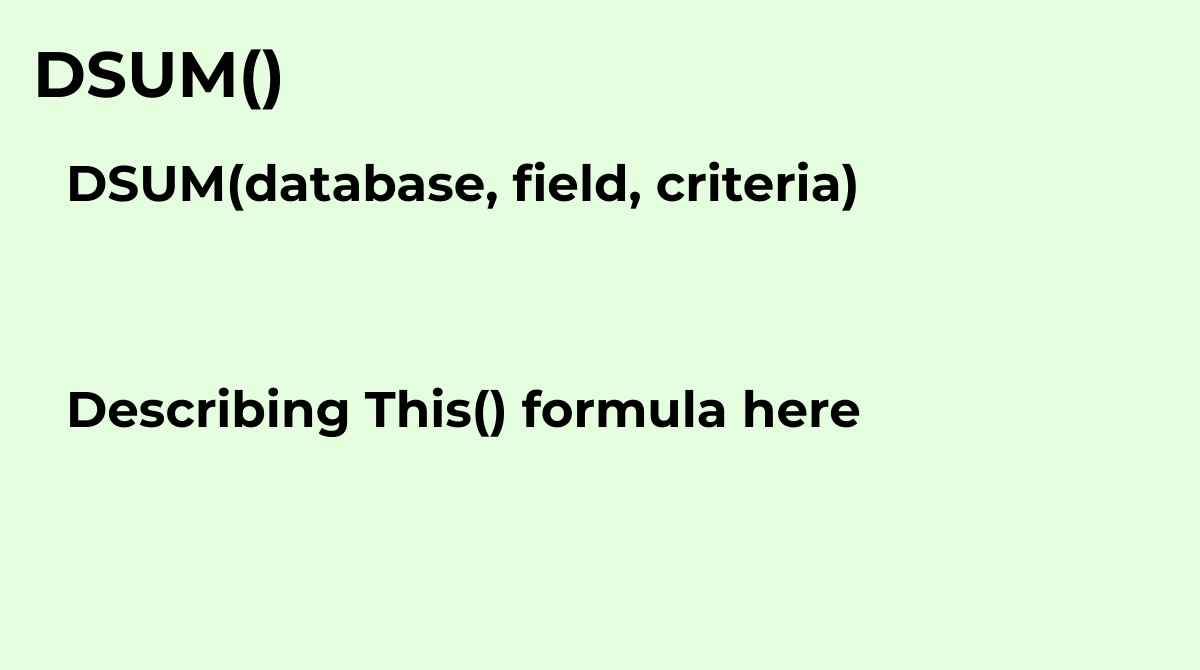Returns the sum of values selected from a database table-like array or range using a SQL-like query.
`DSUM(database, field, criteria)`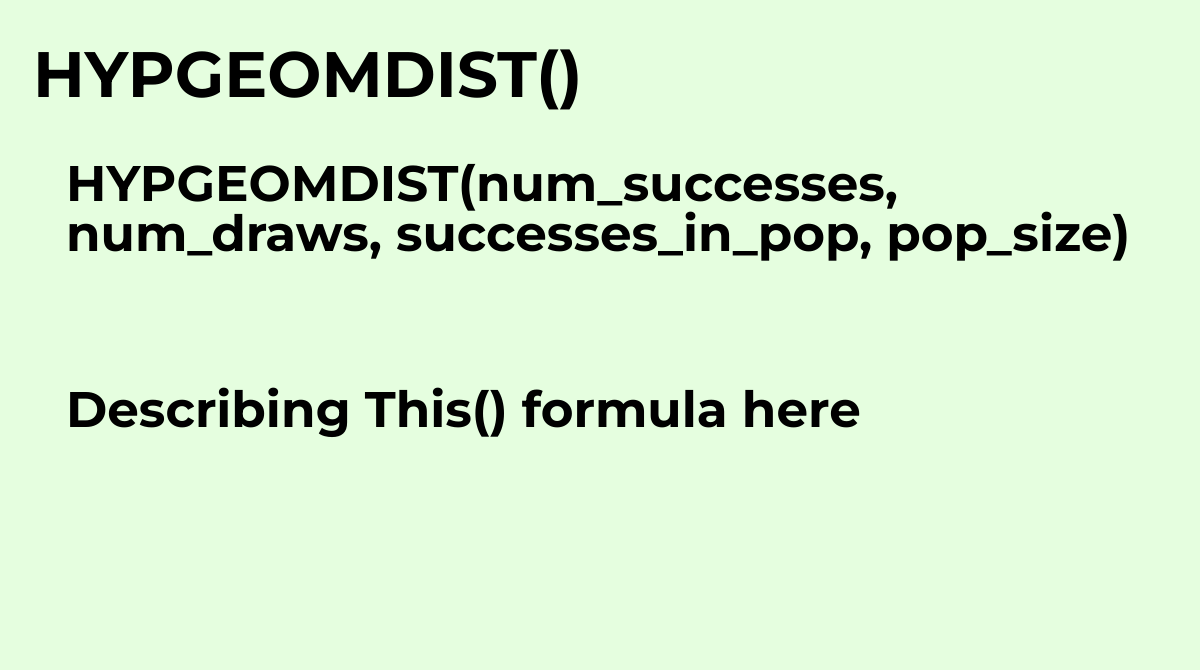Calculates the probability of drawing a certain number of successes in a certain number of tries ...
`HYPGEOMDIST(num_successes, num_draws, successes_in_pop, pop_size)`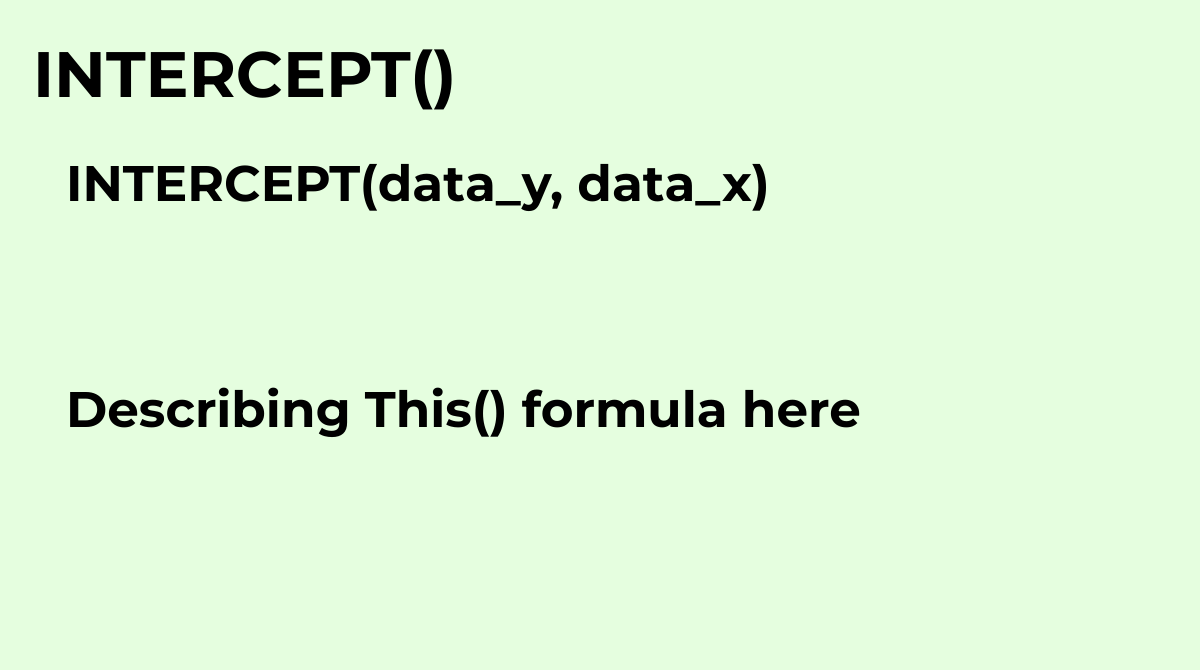Calculates the y-value at which the line resulting from linear regression of a dataset will inter...
`INTERCEPT(data_y, data_x)`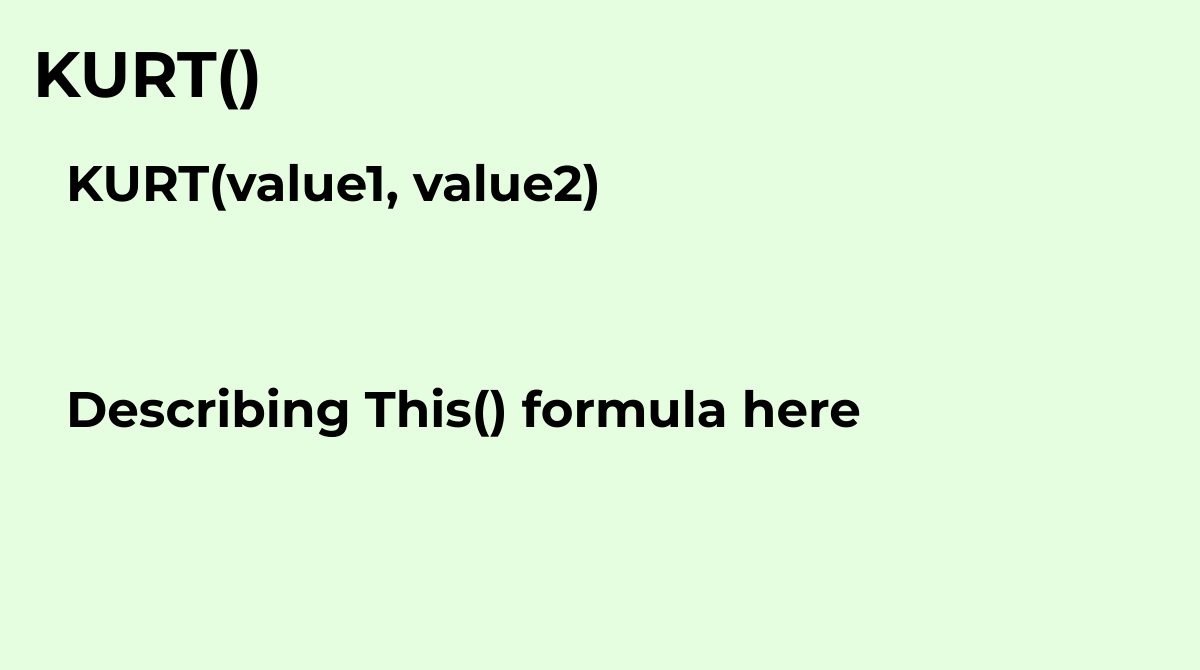Calculates the kurtosis of a dataset, which describes the shape, and in particular the "peakednes...
`KURT(value1, value2)`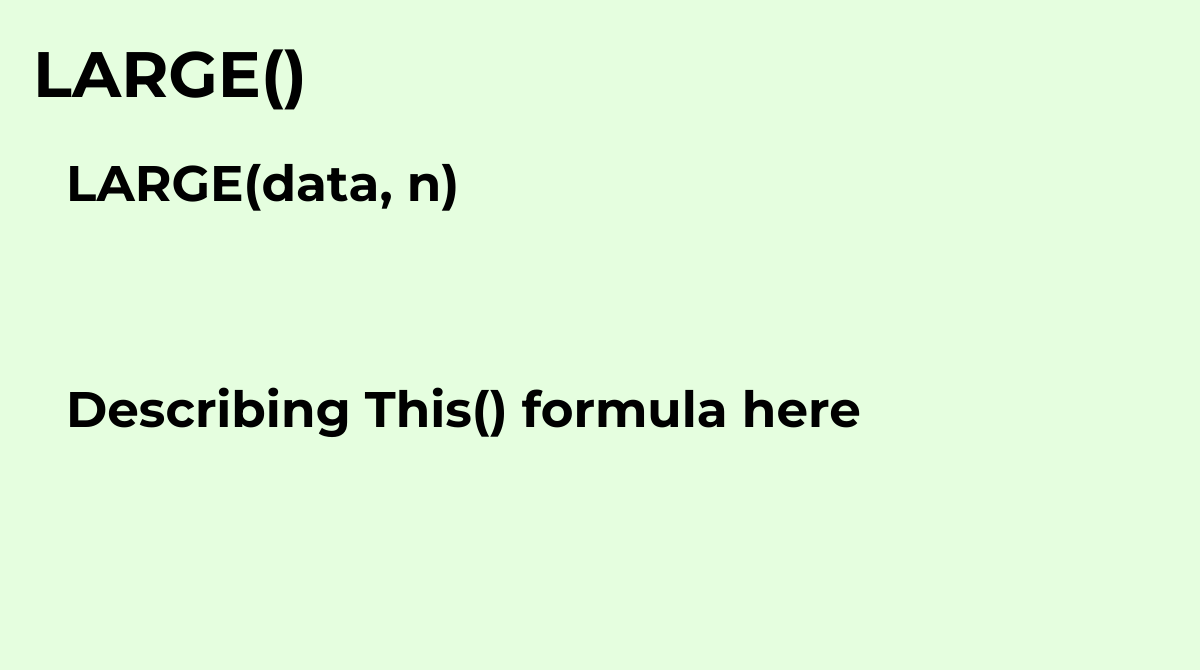Returns the nth largest element from a data set, where n is user-defined.
`LARGE(data, n)`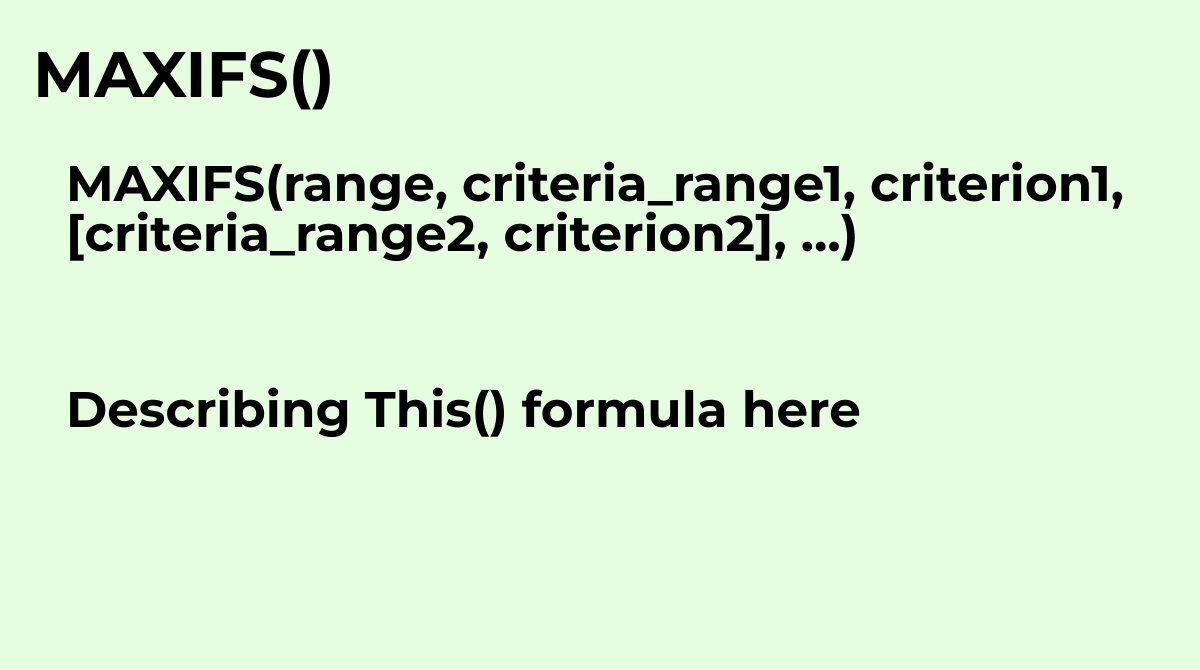Returns the maximum value in a range of cells, filtered by a set of criteria..
`MAXIFS(range, criteria_range1, criterion1, [criteria_range2, criterion2], …)`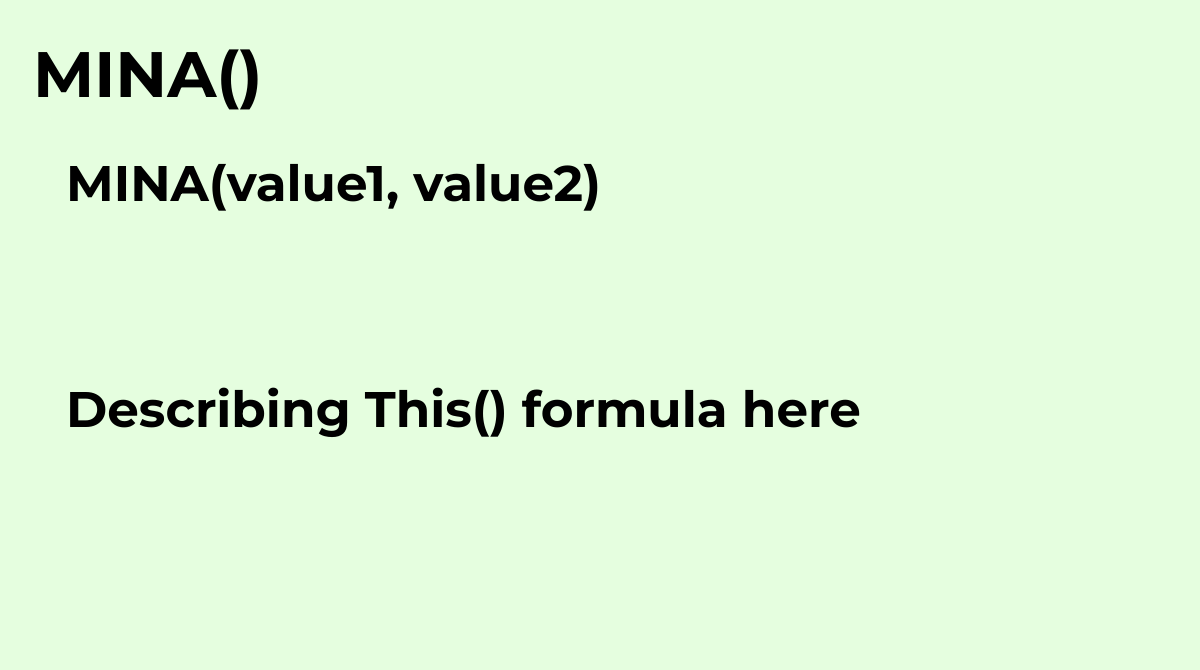Returns the minimum numeric value in a dataset.
`MINA(value1, value2)`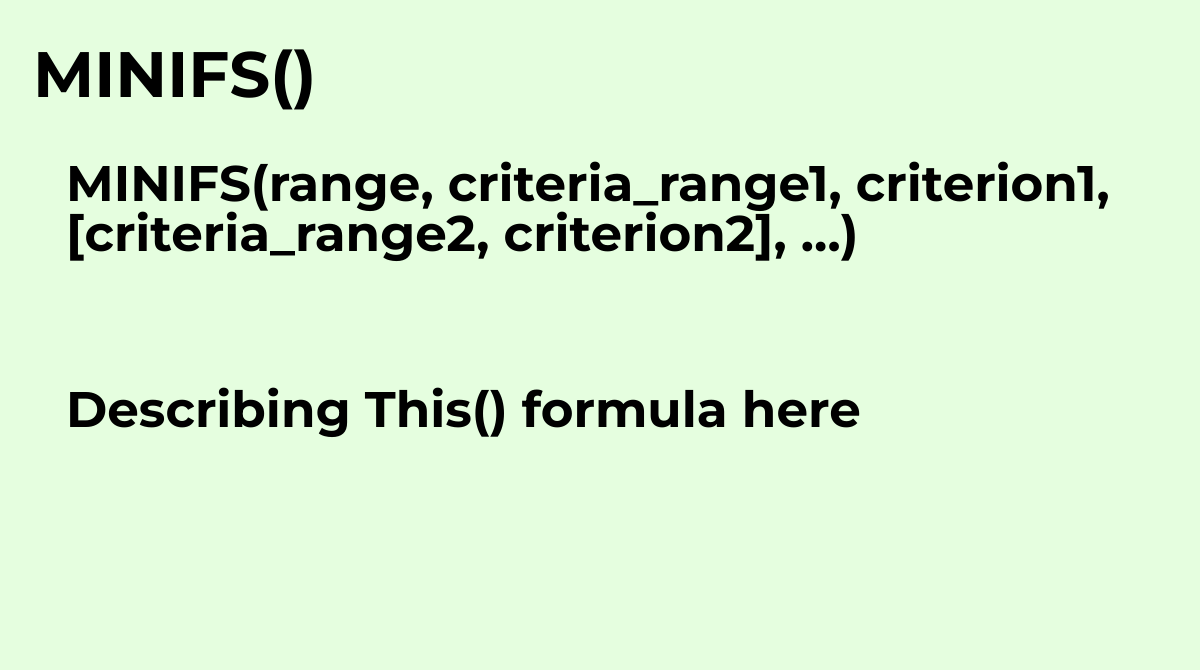Returns the minimum value in a range of cells, filtered by a set of criteria..
`MINIFS(range, criteria_range1, criterion1, [criteria_range2, criterion2], …)`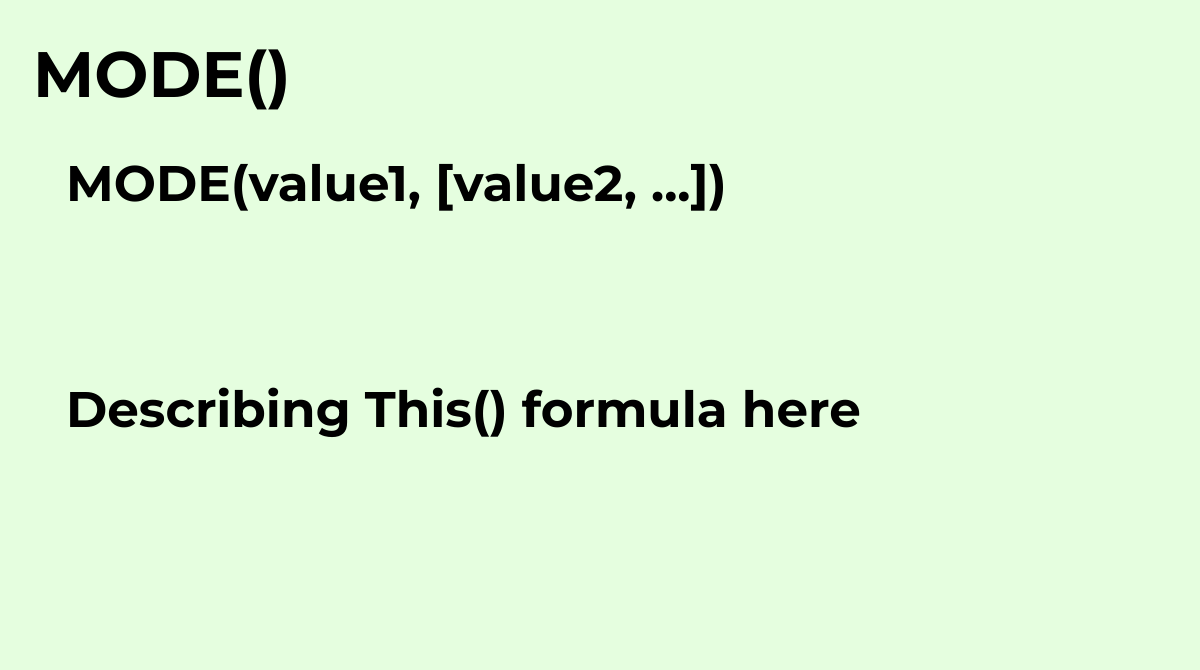Returns the most commonly occurring value in a dataset.
`MODE(value1, [value2, ...])`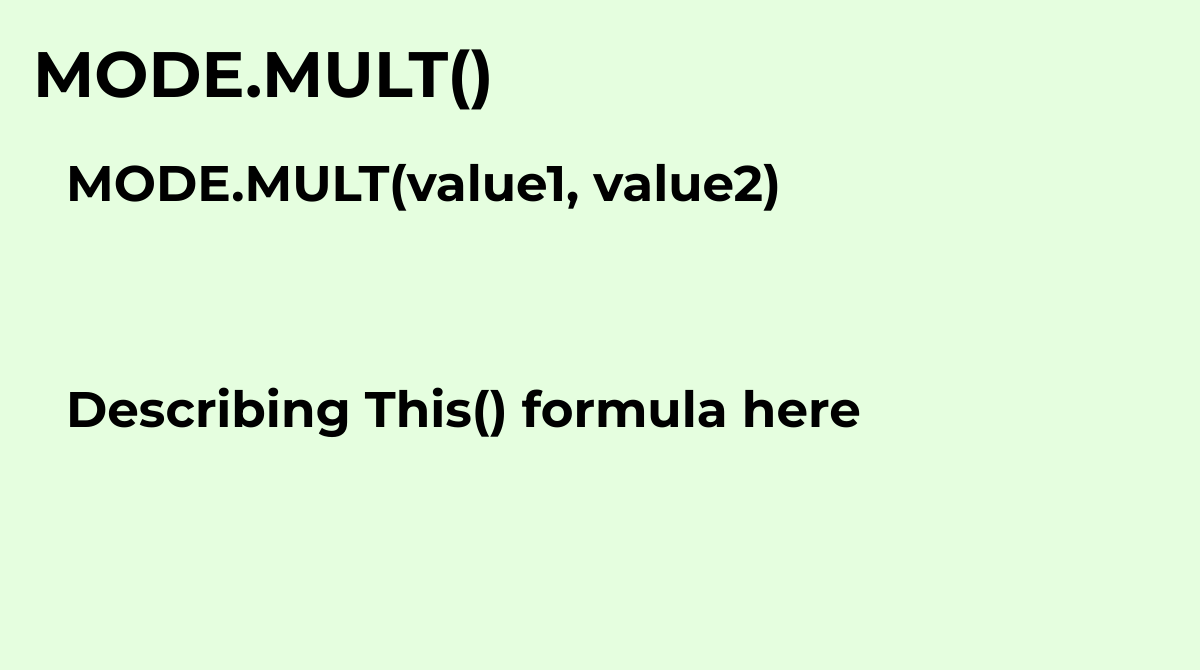Returns the most commonly occurring values in a dataset..
`MODE.MULT(value1, value2)`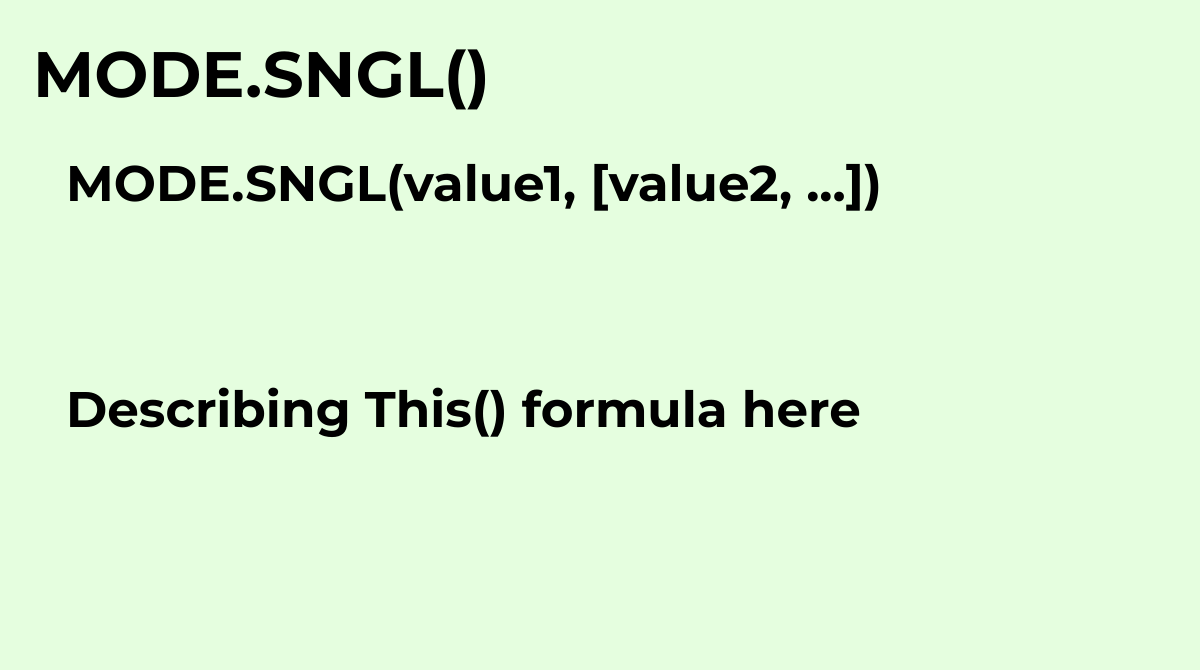See MODE
`MODE.SNGL(value1, [value2, ...])`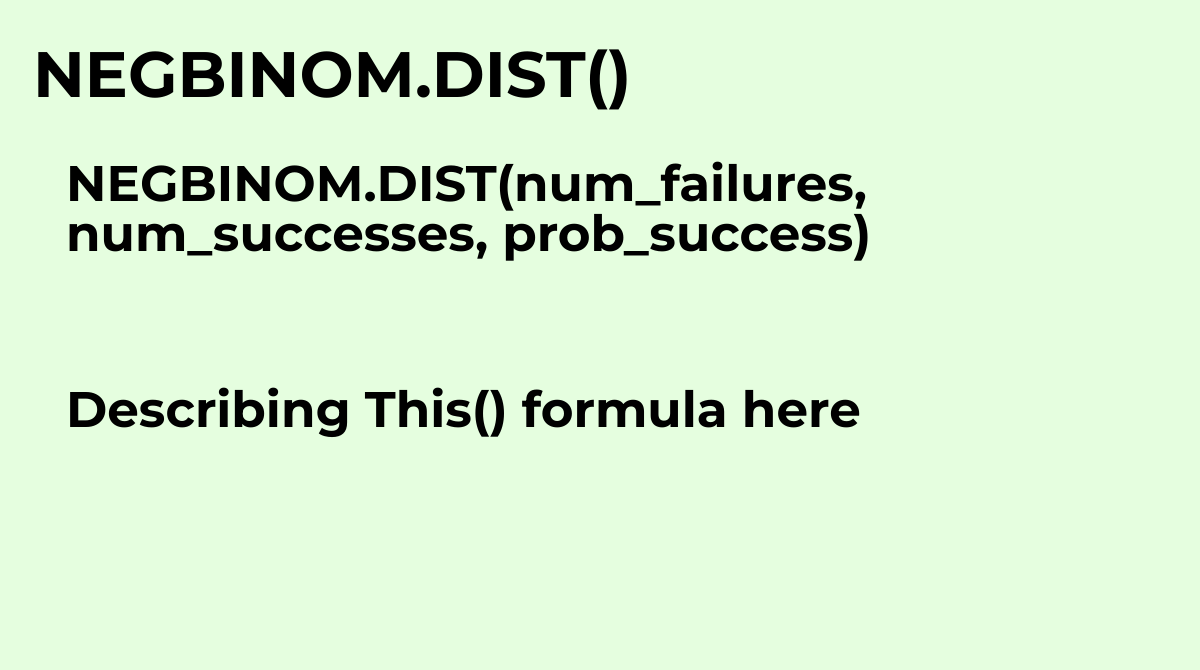See NEGBINOMDIST
`NEGBINOM.DIST(num_failures, num_successes, prob_success)`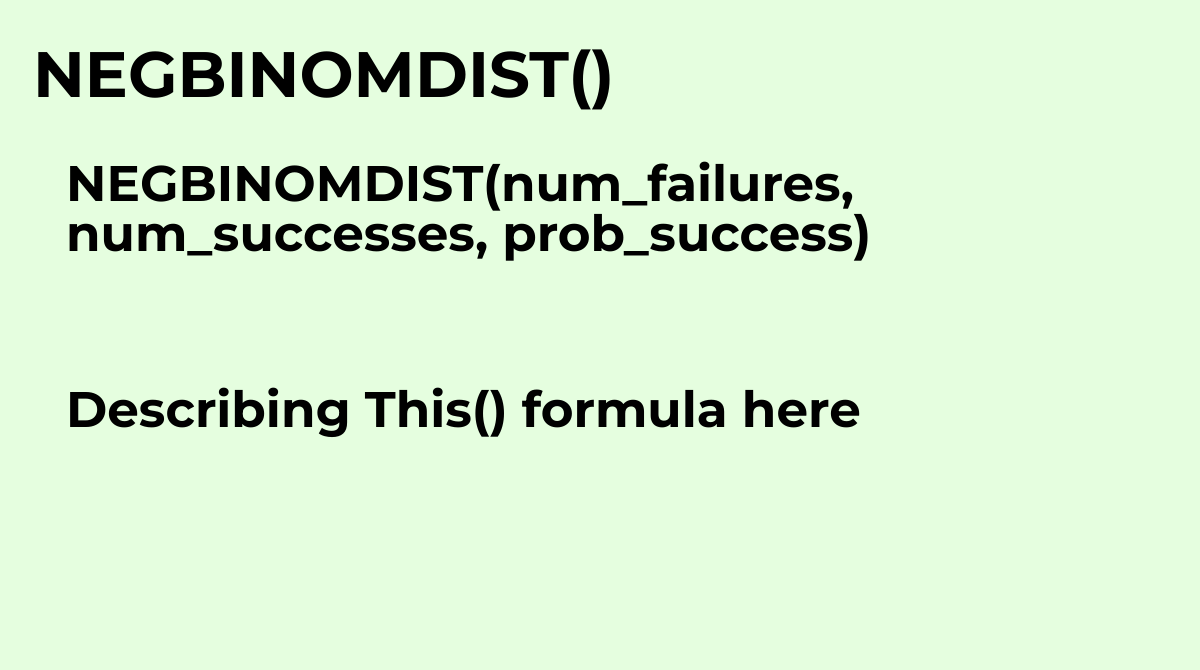Calculates the probability of drawing a certain number of failures before a certain number of suc...
`NEGBINOMDIST(num_failures, num_successes, prob_success)`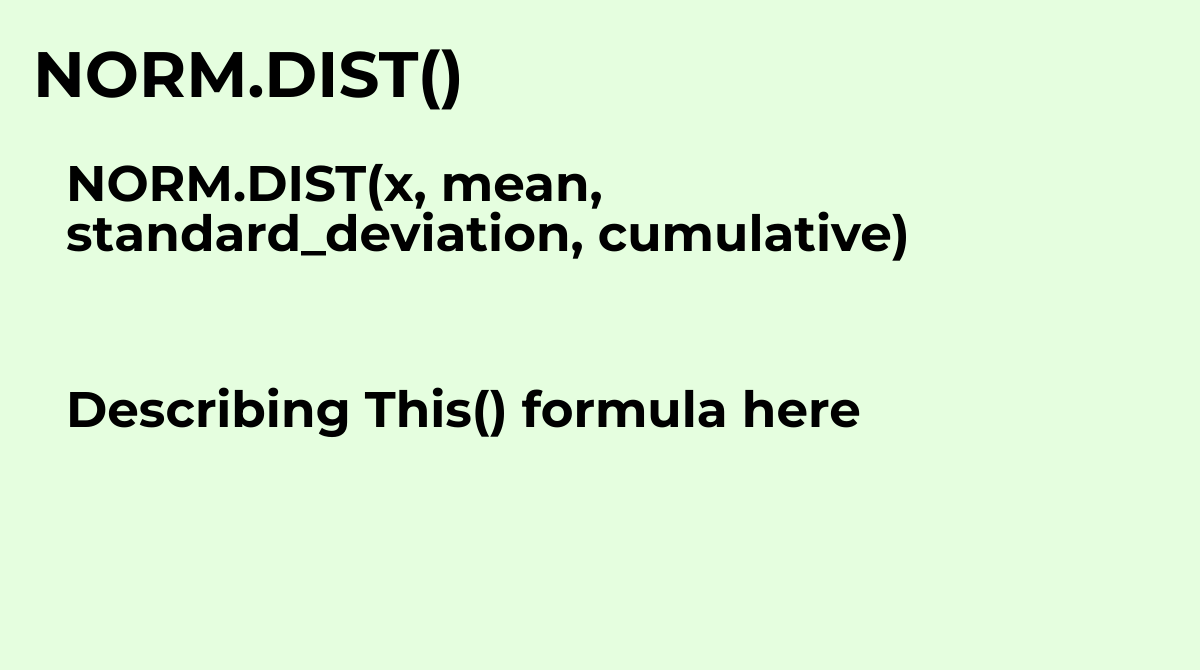See NORMDIST
`NORM.DIST(x, mean, standard_deviation, cumulative)`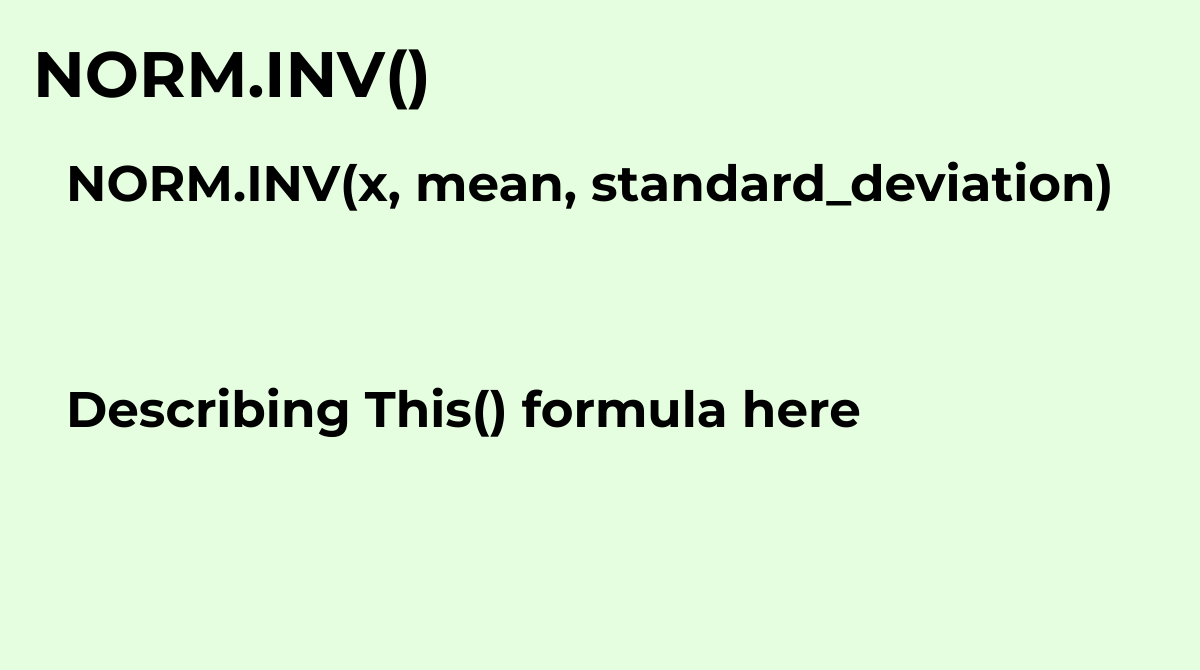See NORMINV
`NORM.INV(x, mean, standard_deviation)`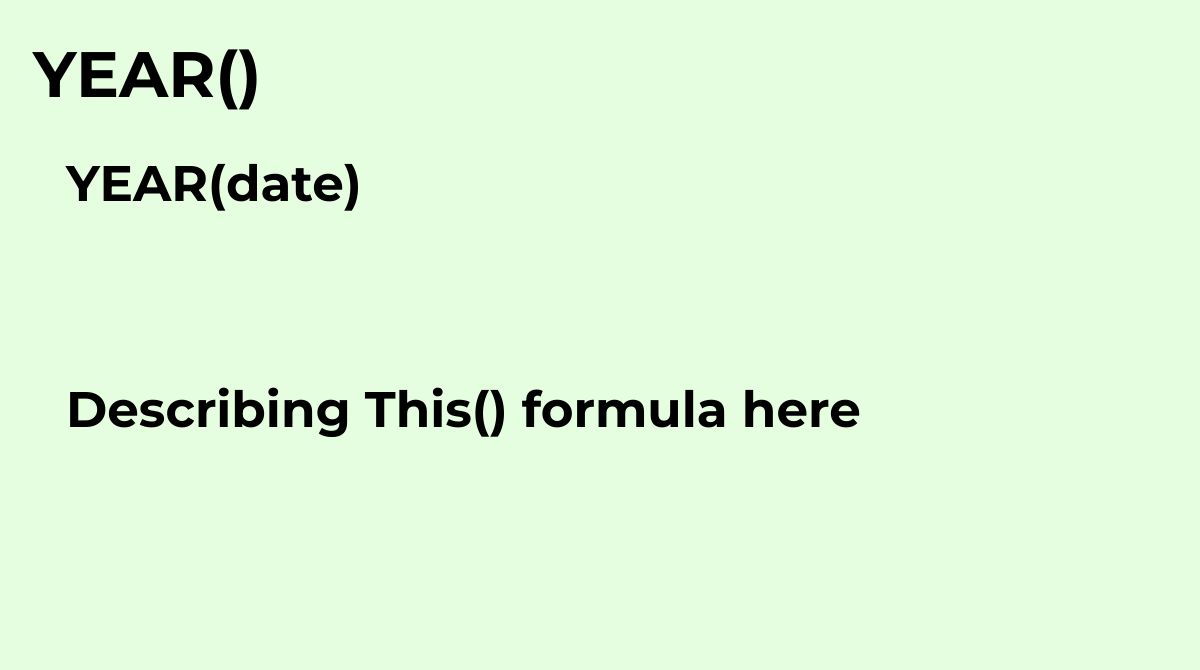Returns the year specified by a given date.Common questions about the YEAR formula:What is the sy...
`YEAR(date)`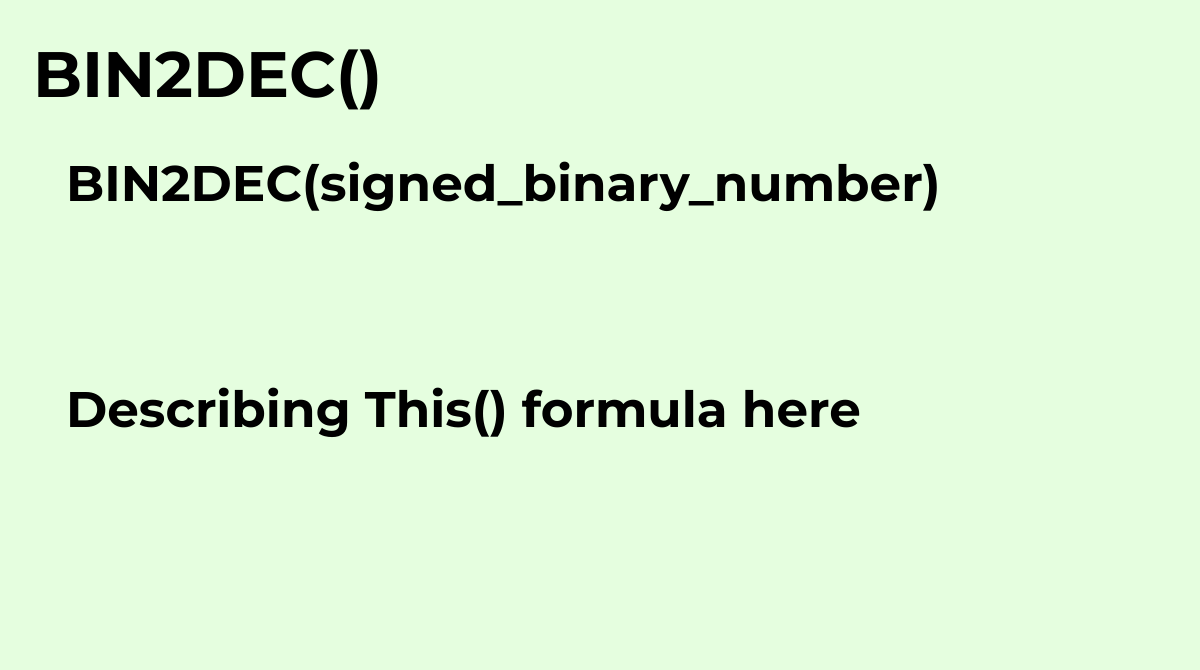Converts a signed binary number to decimal format.
`BIN2DEC(signed_binary_number)`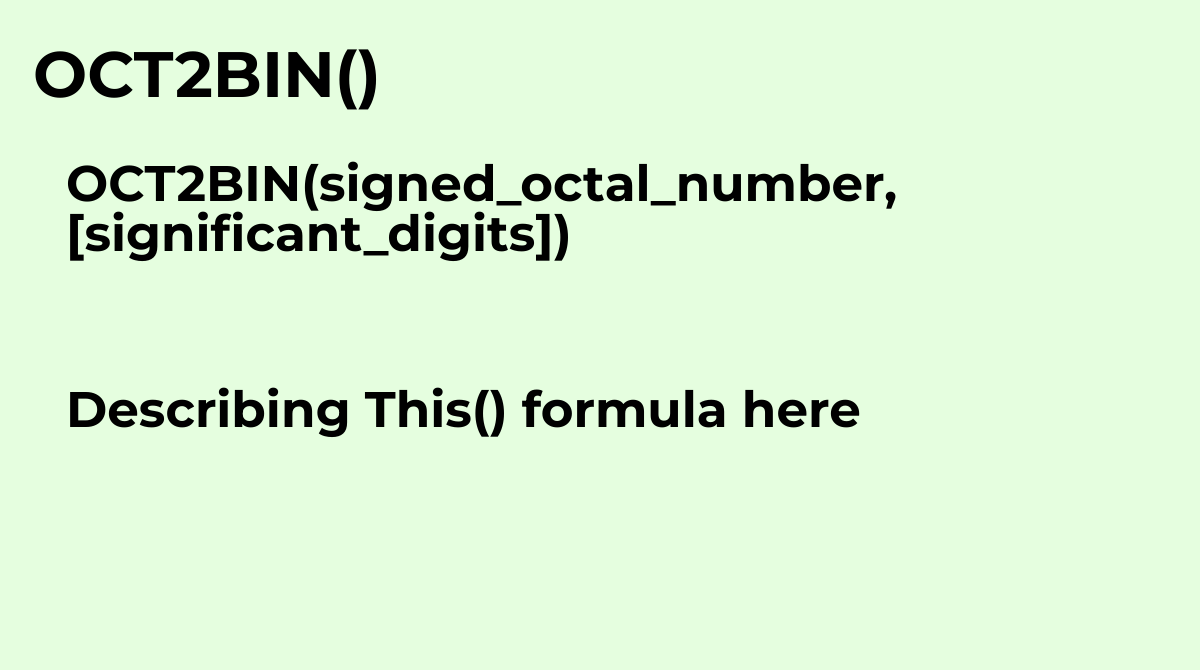Converts a signed octal number to signed binary format.
`OCT2BIN(signed_octal_number, [significant_digits])`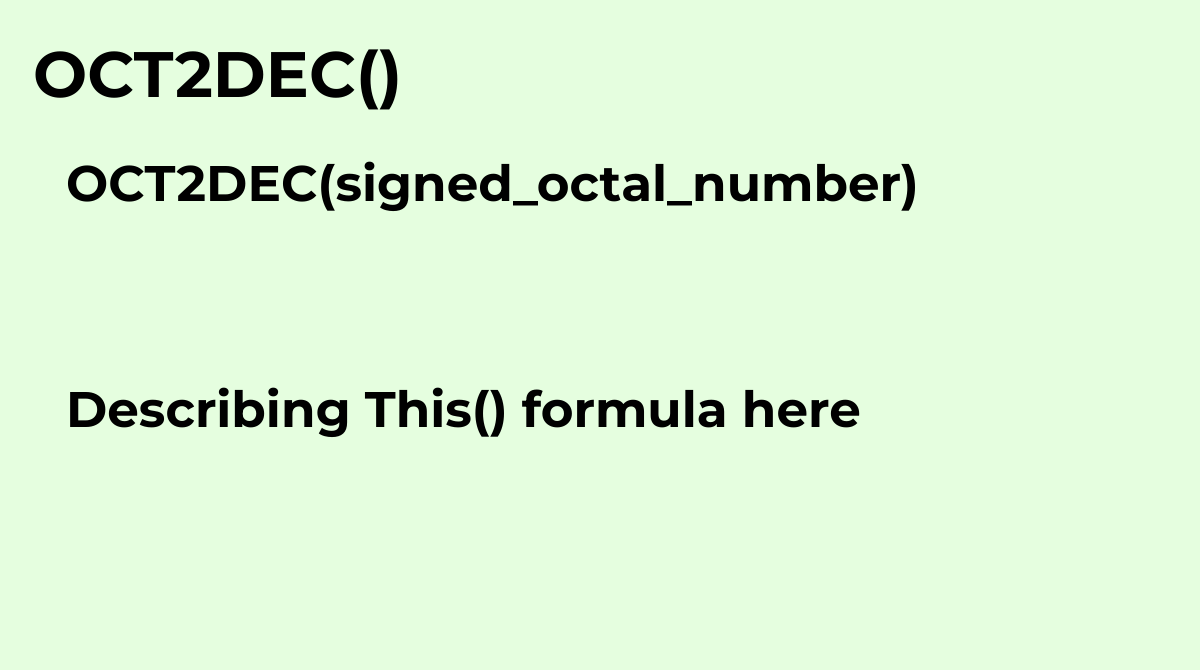Converts a signed octal number to decimal format.
`OCT2DEC(signed_octal_number)`

Displaying items 289-336 of 515 in total